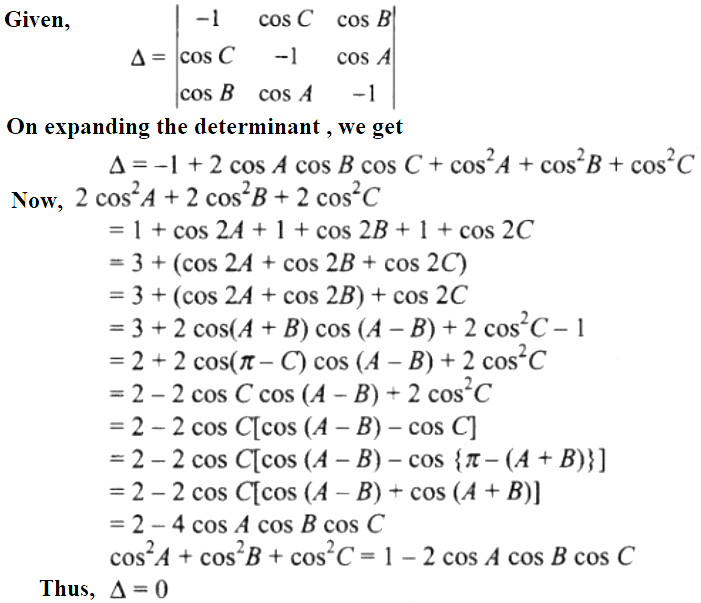# NCERT Exemplar Solutions for Class 12 Maths Chapter 4 Determinants

If students are looking for a resource to learn concepts in a better way and also practise and prepare for their exams, the NCERT Exemplar textbooks are all that one needs. Along with the Exemplar textbooks, students can now access NCERT Exemplar Solutions, available subject-wise, to clear any doubts regarding the concepts and problems in the textbooks. All solutions are created following the latest CBSE patterns to help students score better marks.

The 4th Chapter of NCERT Exemplar Solutions for Class 12 Mathematics is Determinants. This chapter covers topics such as introduction to determinants, a determinant matrix of order one, two and three, properties of the determinant, minors and cofactor, adjoint and the inverse of a matrix, applications of matrices and solution of the system of linear equations using the inverse of a matrix. Further, students can make use of the solutions PDF of NCERT Exemplar Solutions for Class 12 Maths Chapter 4 Determinants from the links given below to learn and understand the concepts of determinants in an easy manner.

## Download the PDF of NCERT Exemplar Solutions for Class 12 Maths Chapter 4 Determinants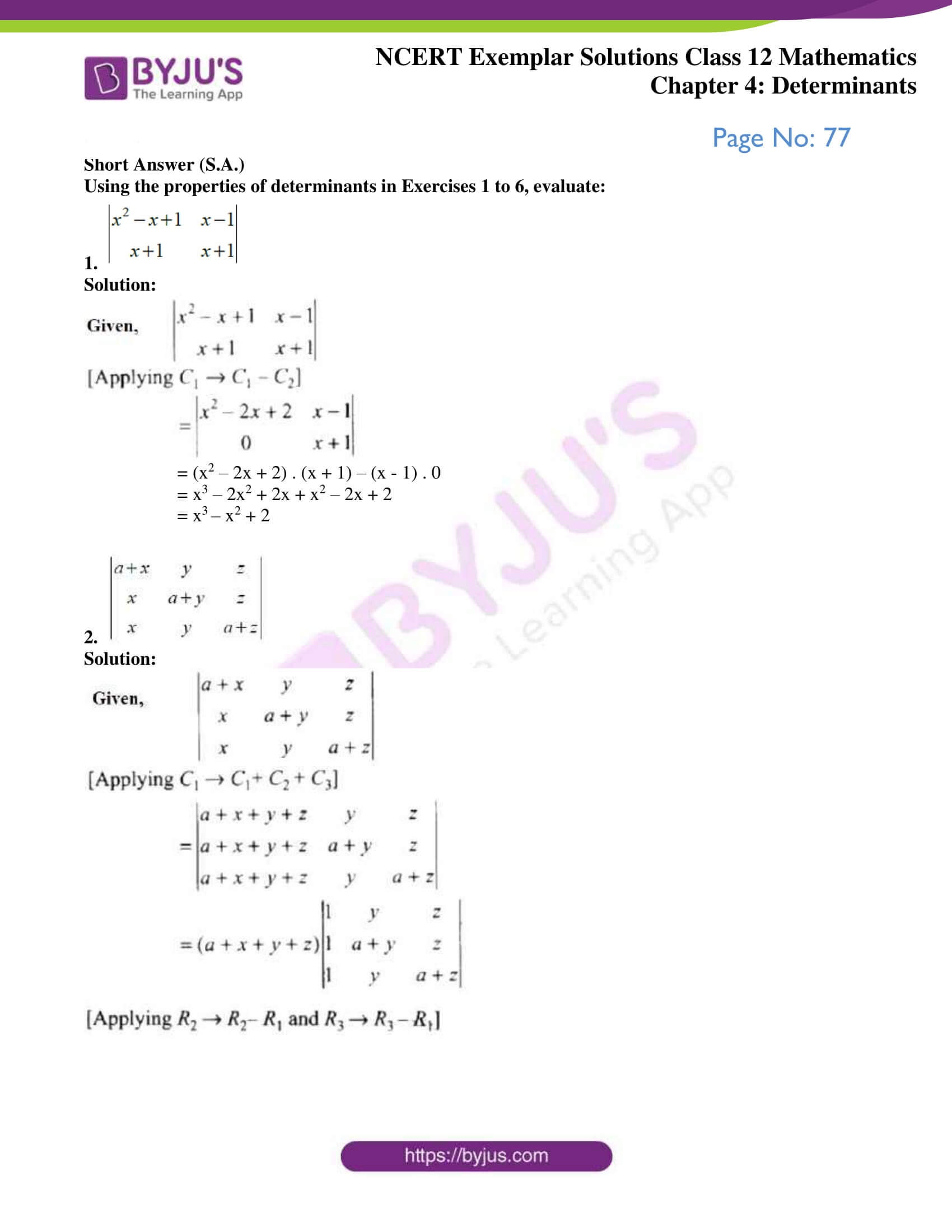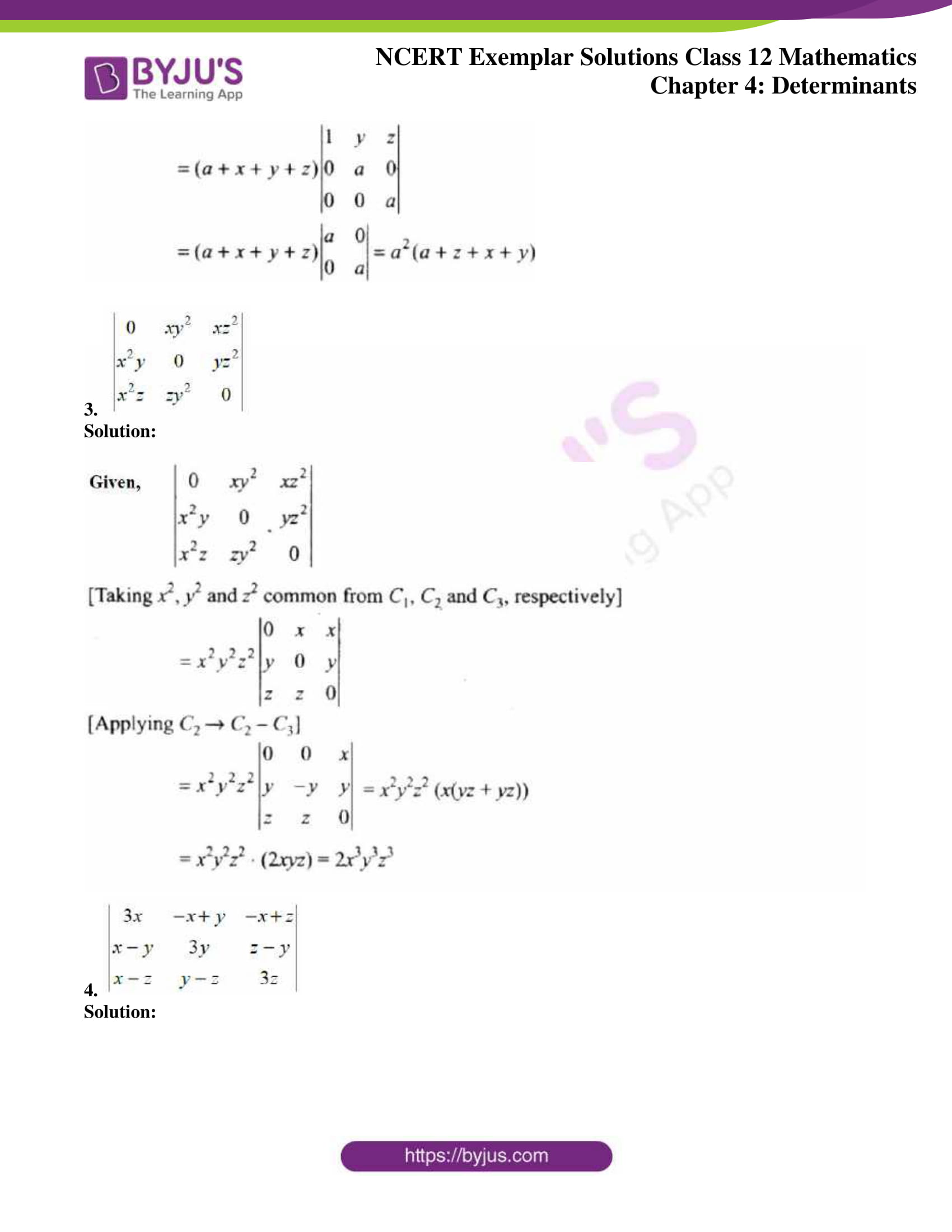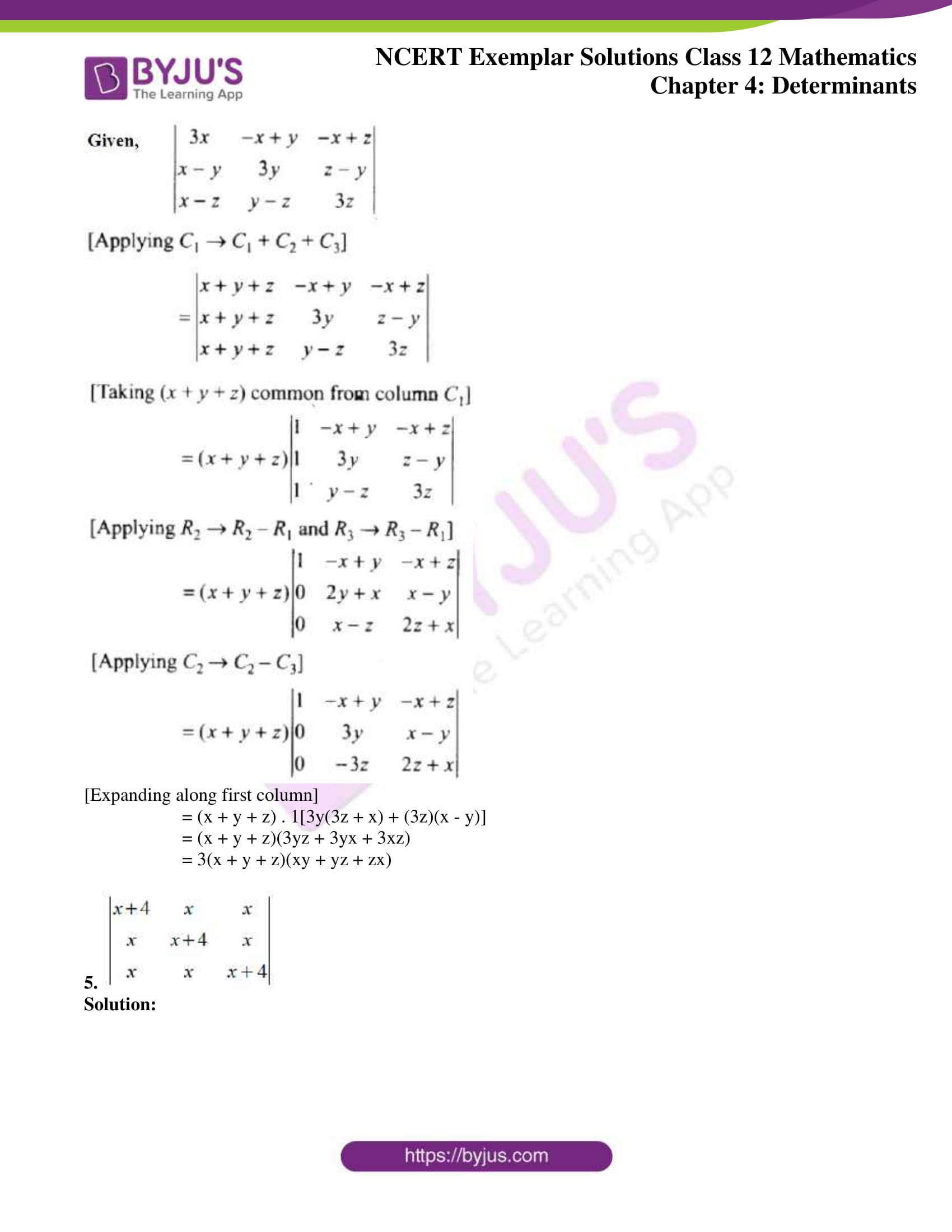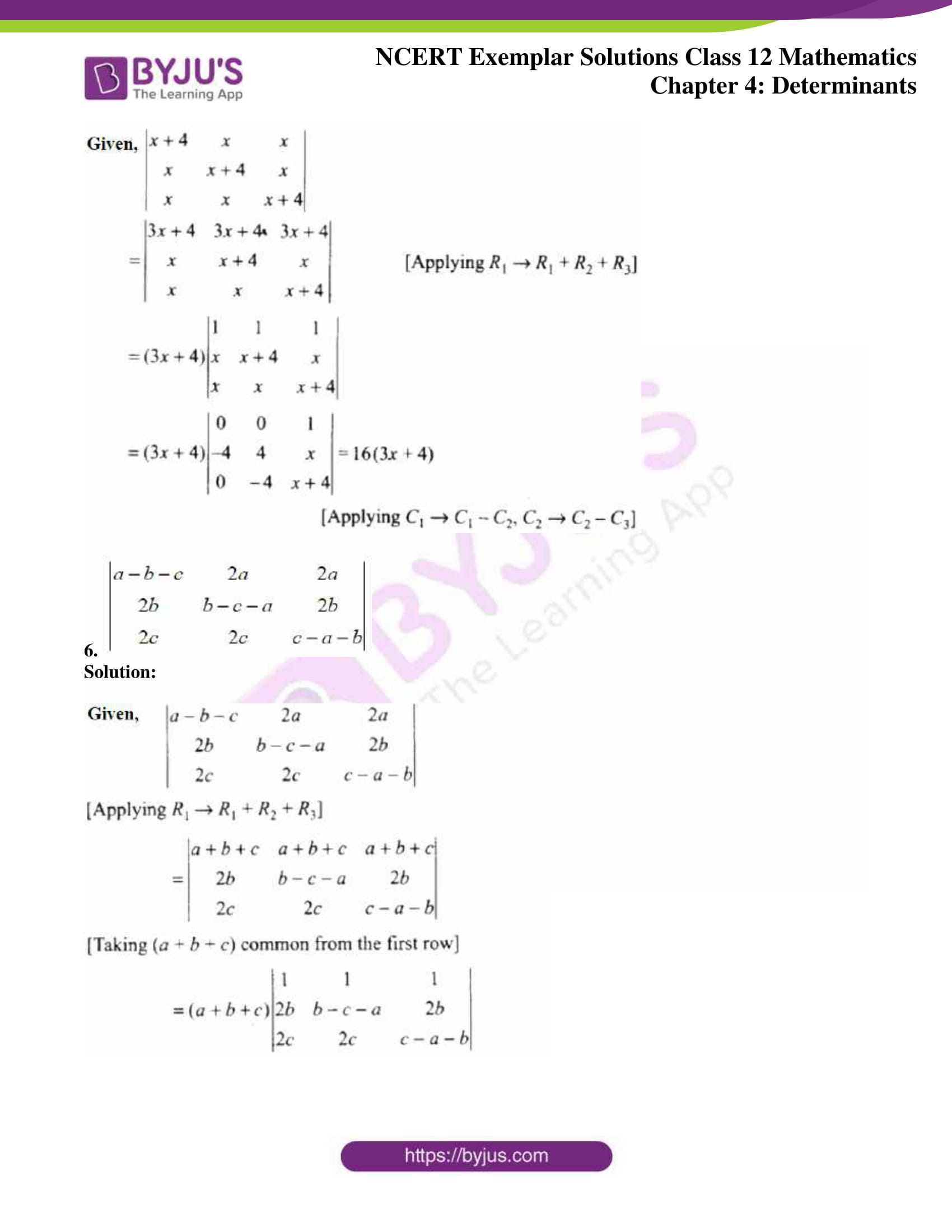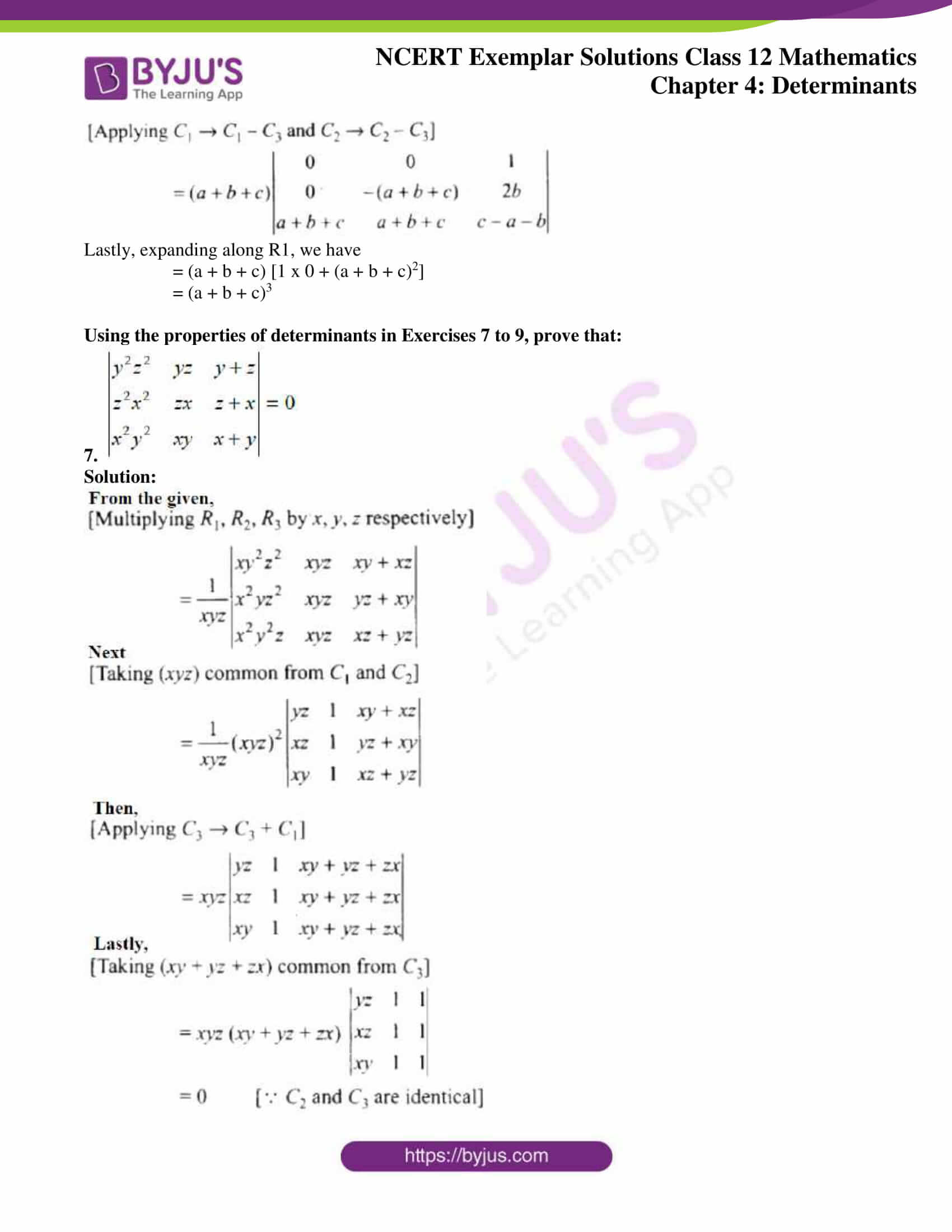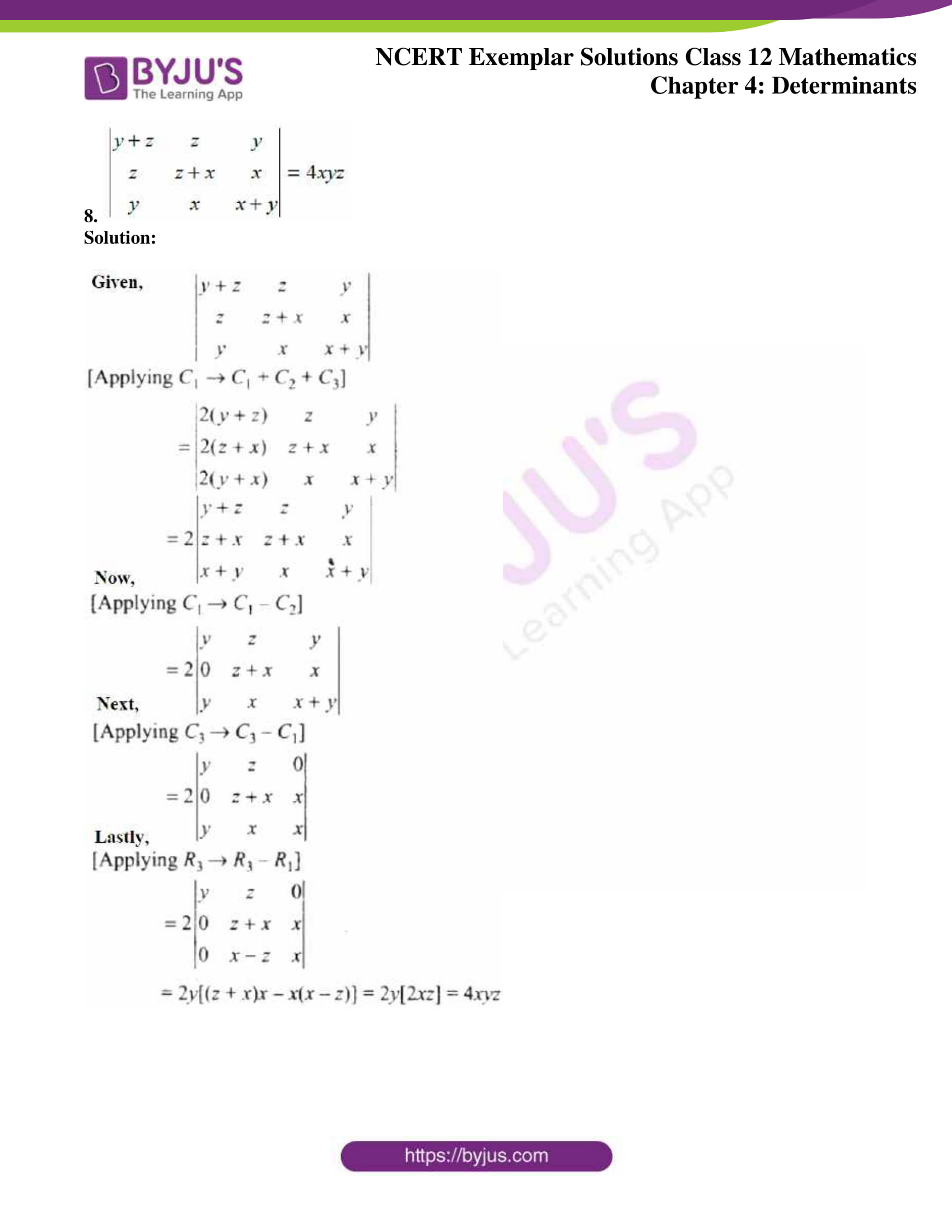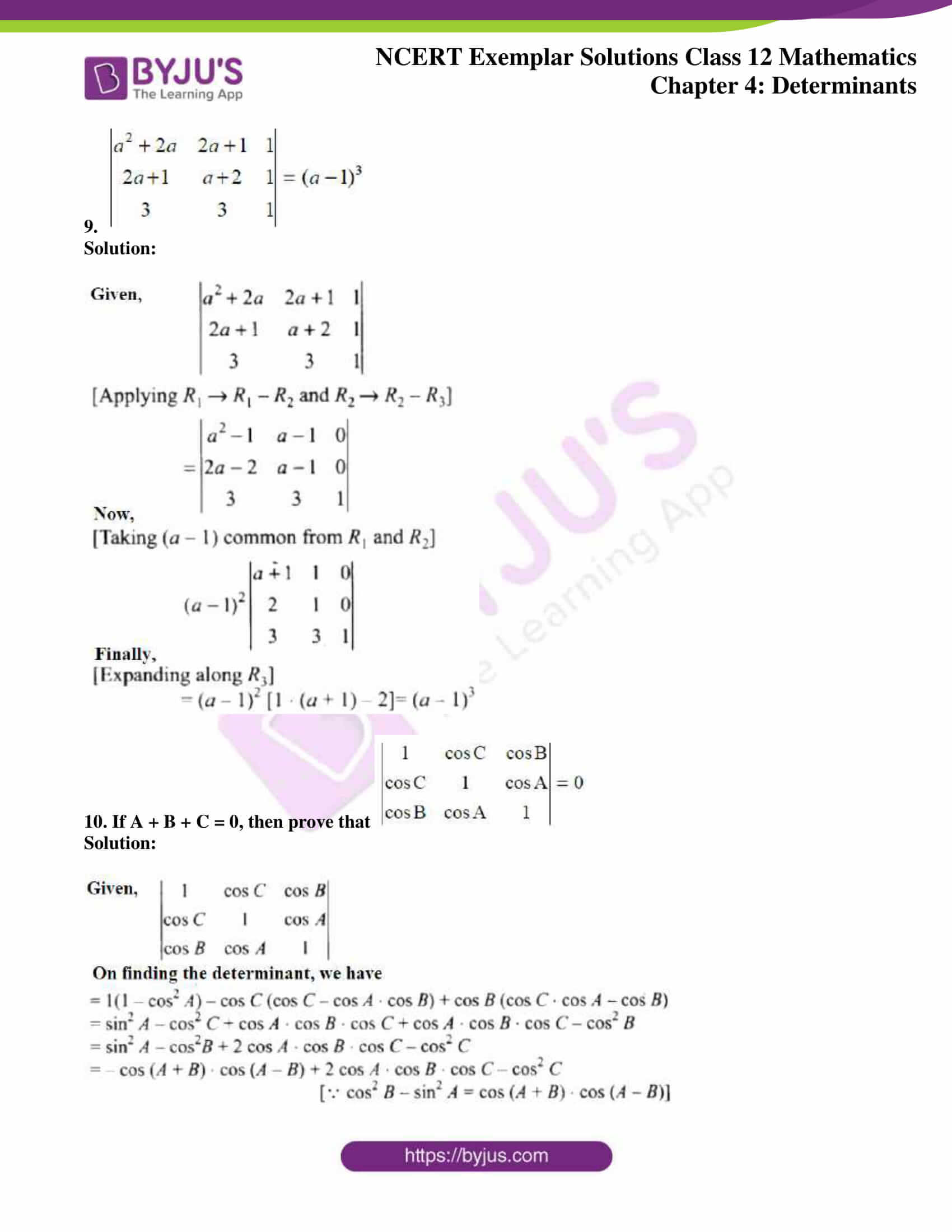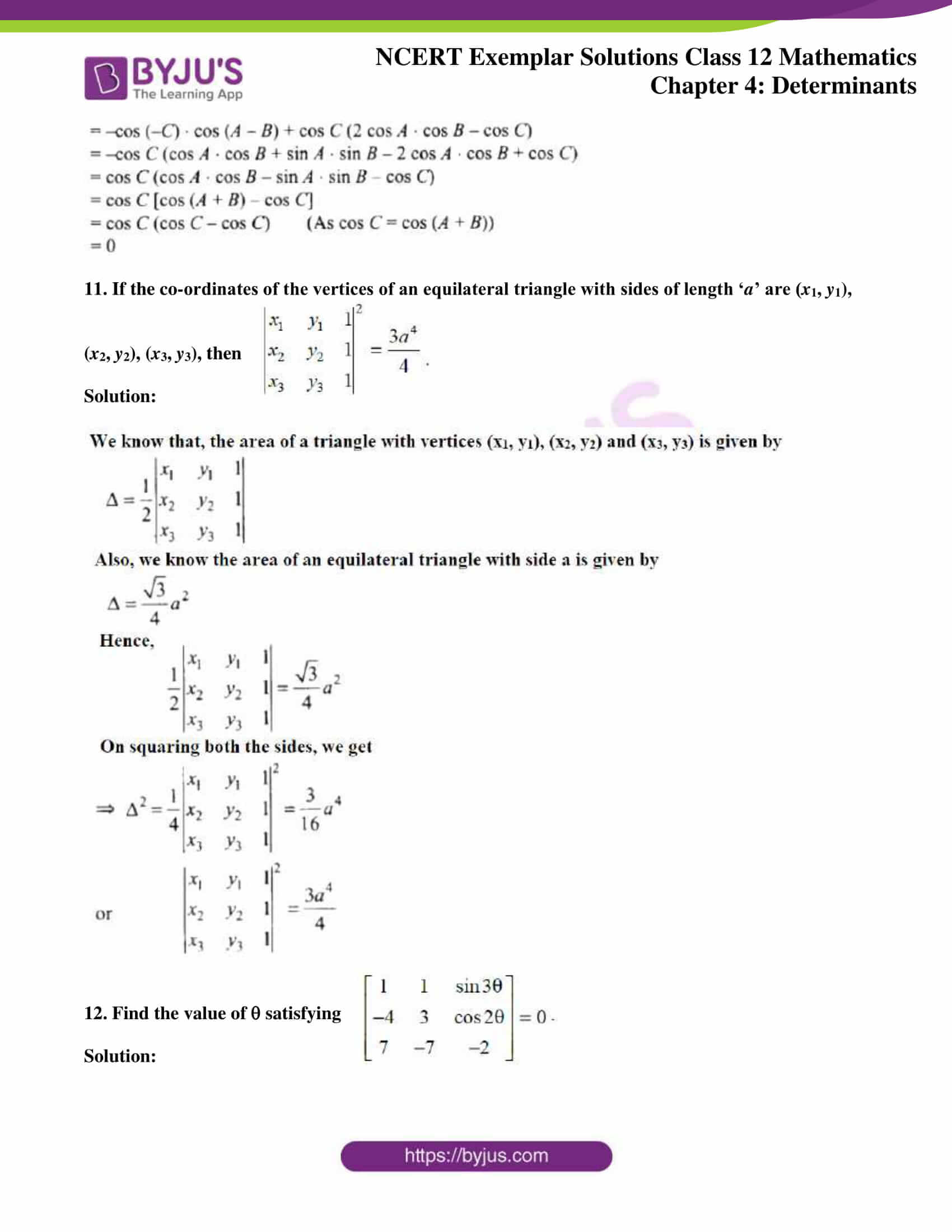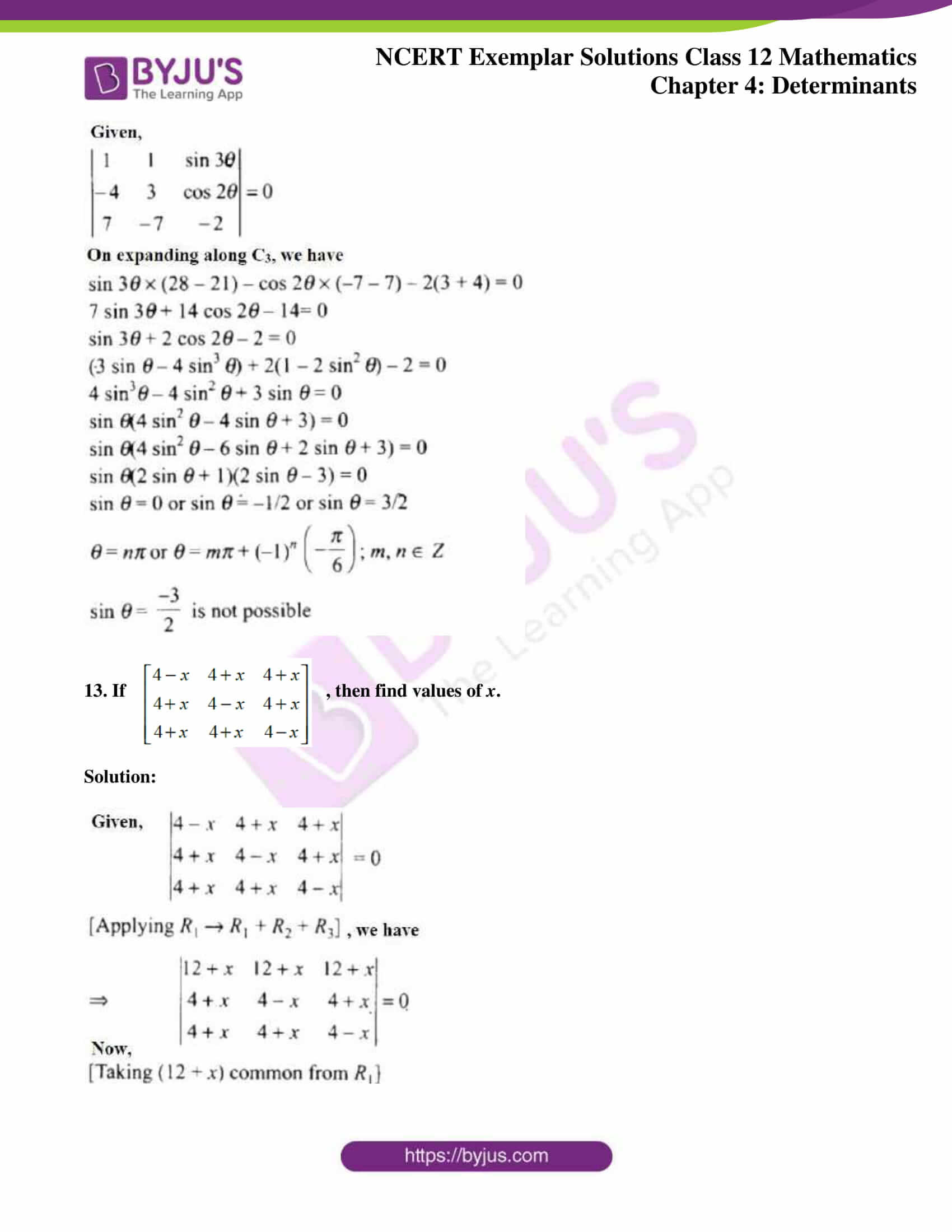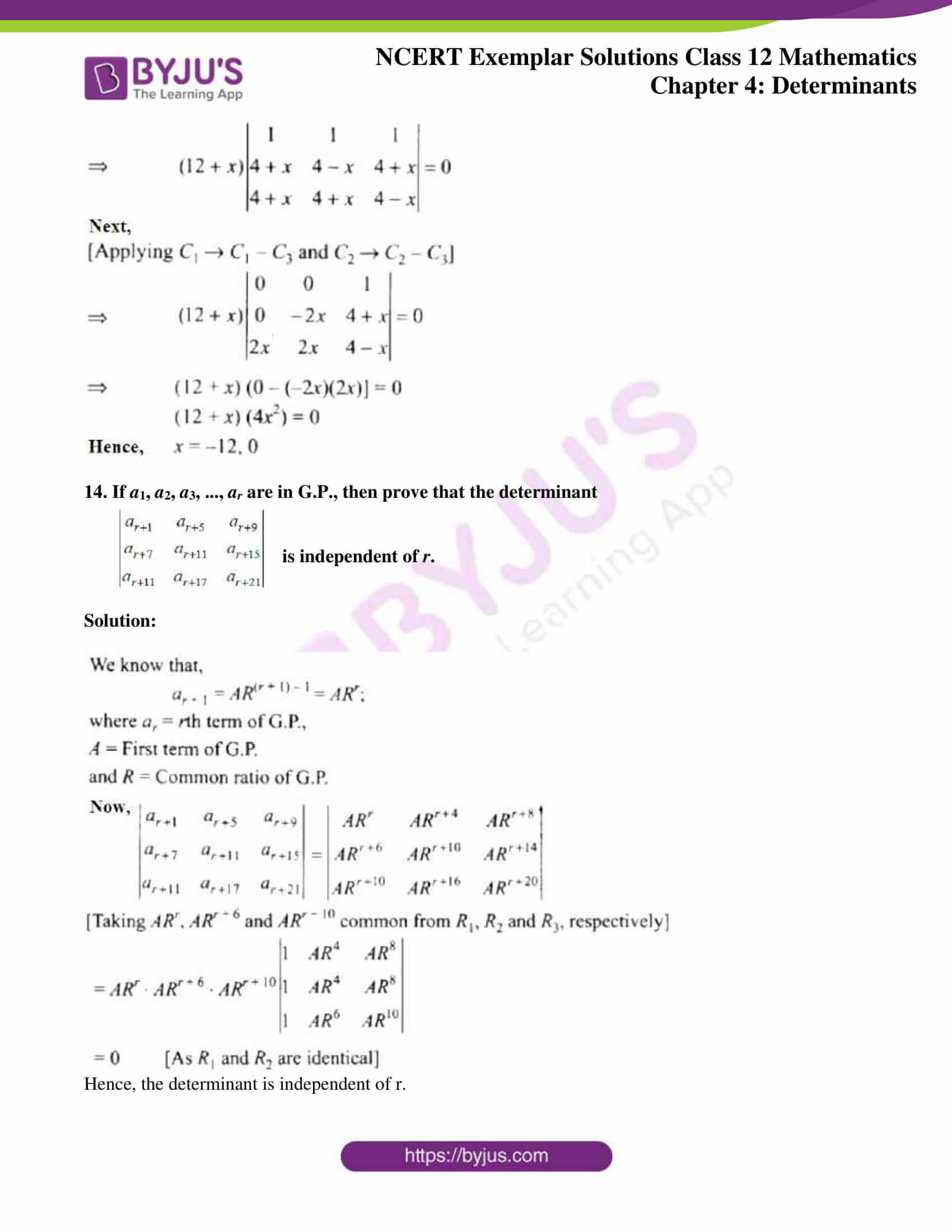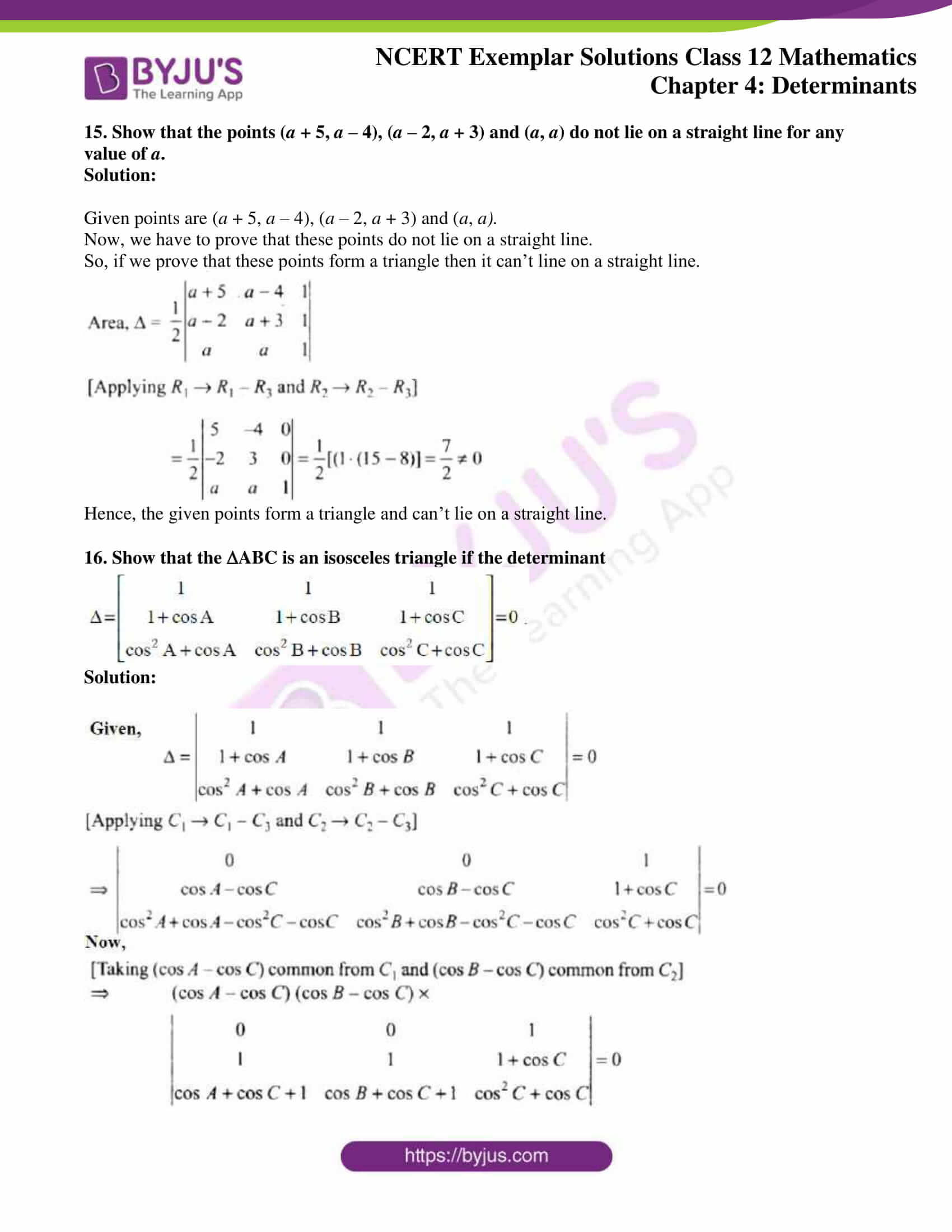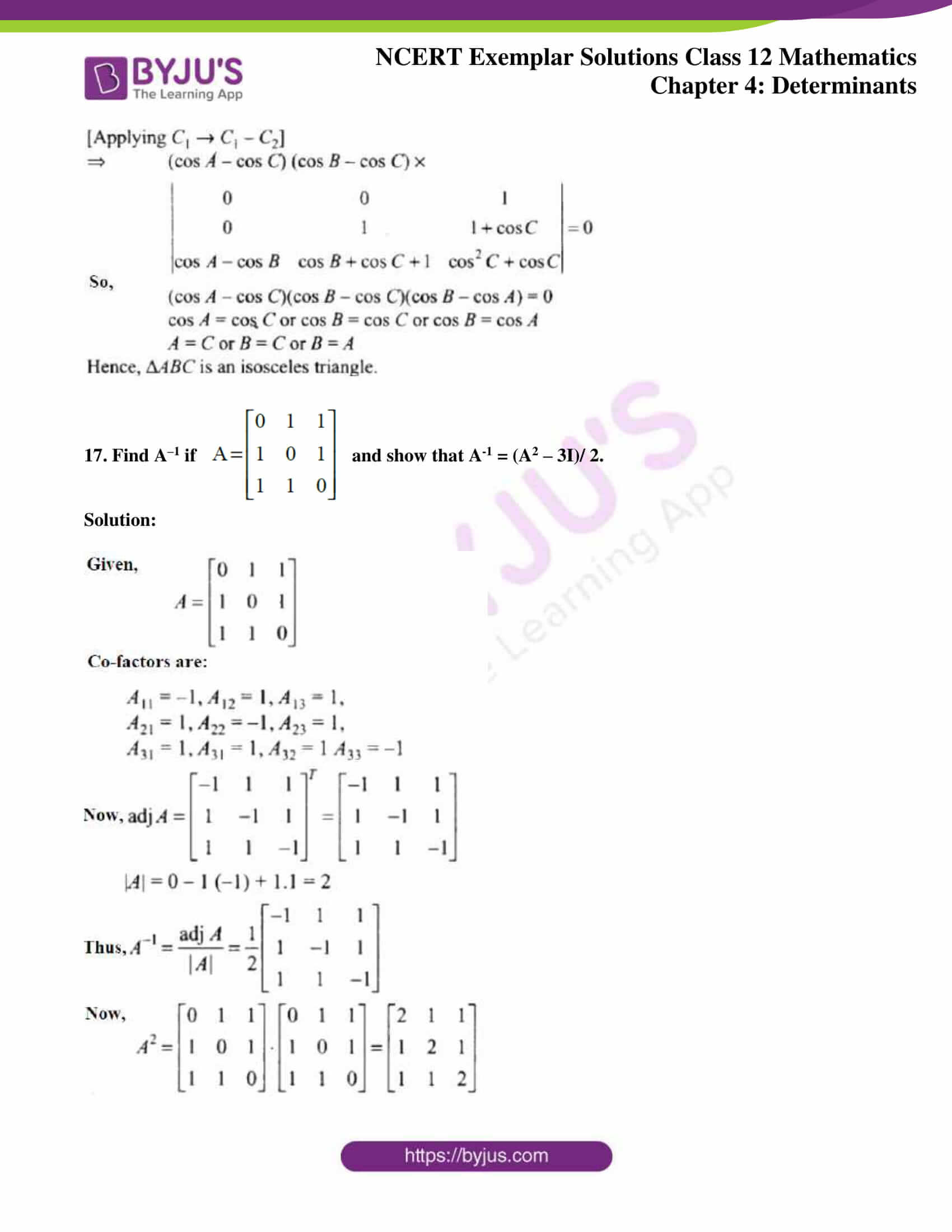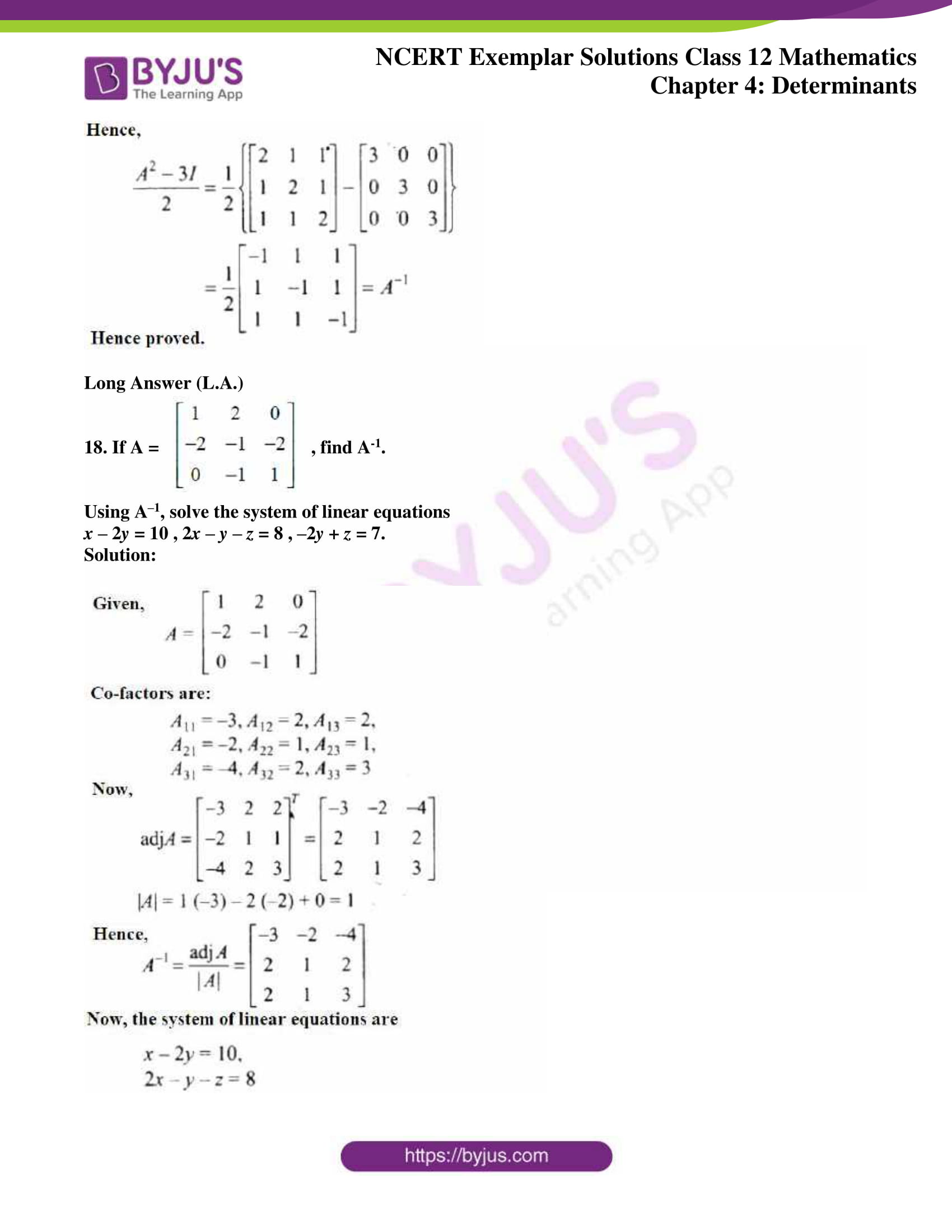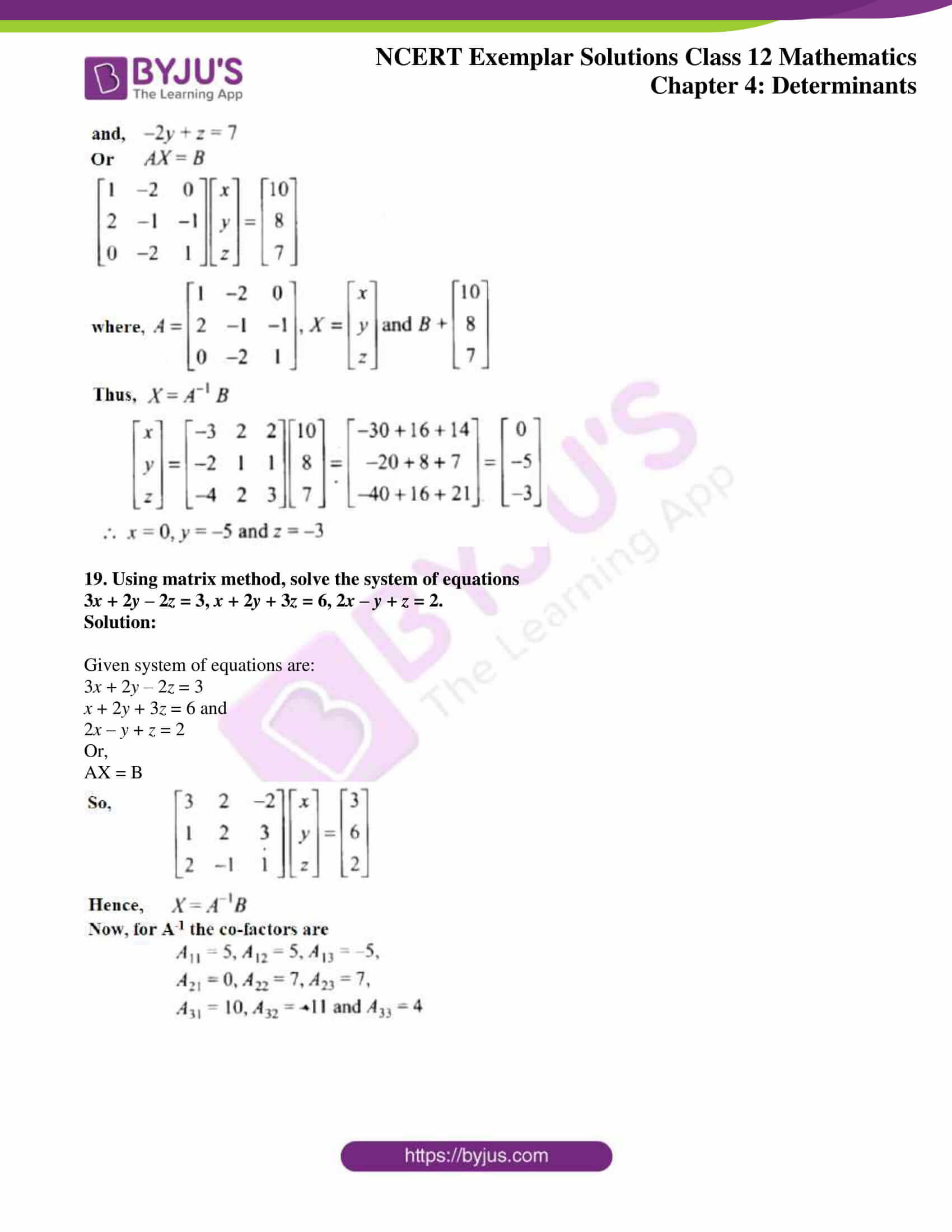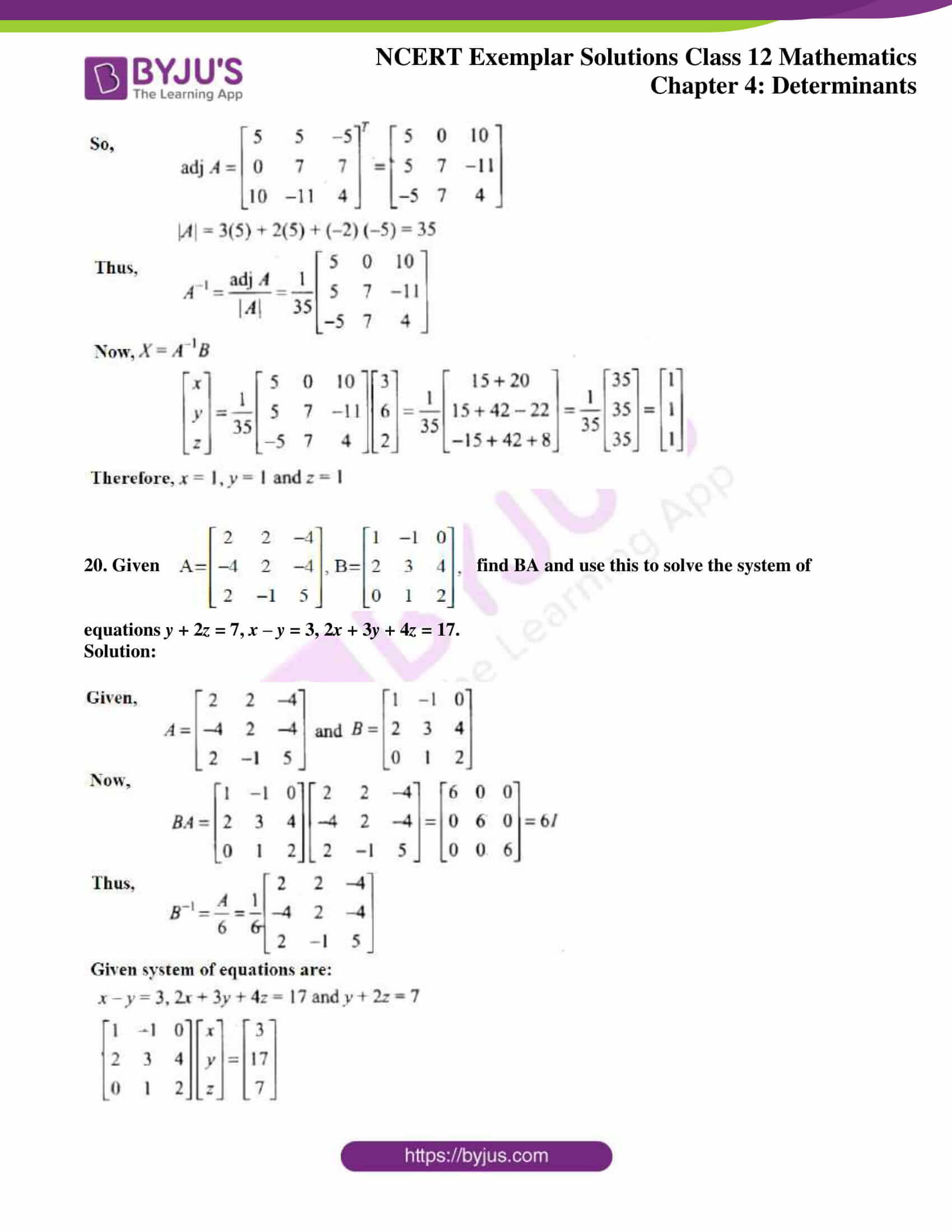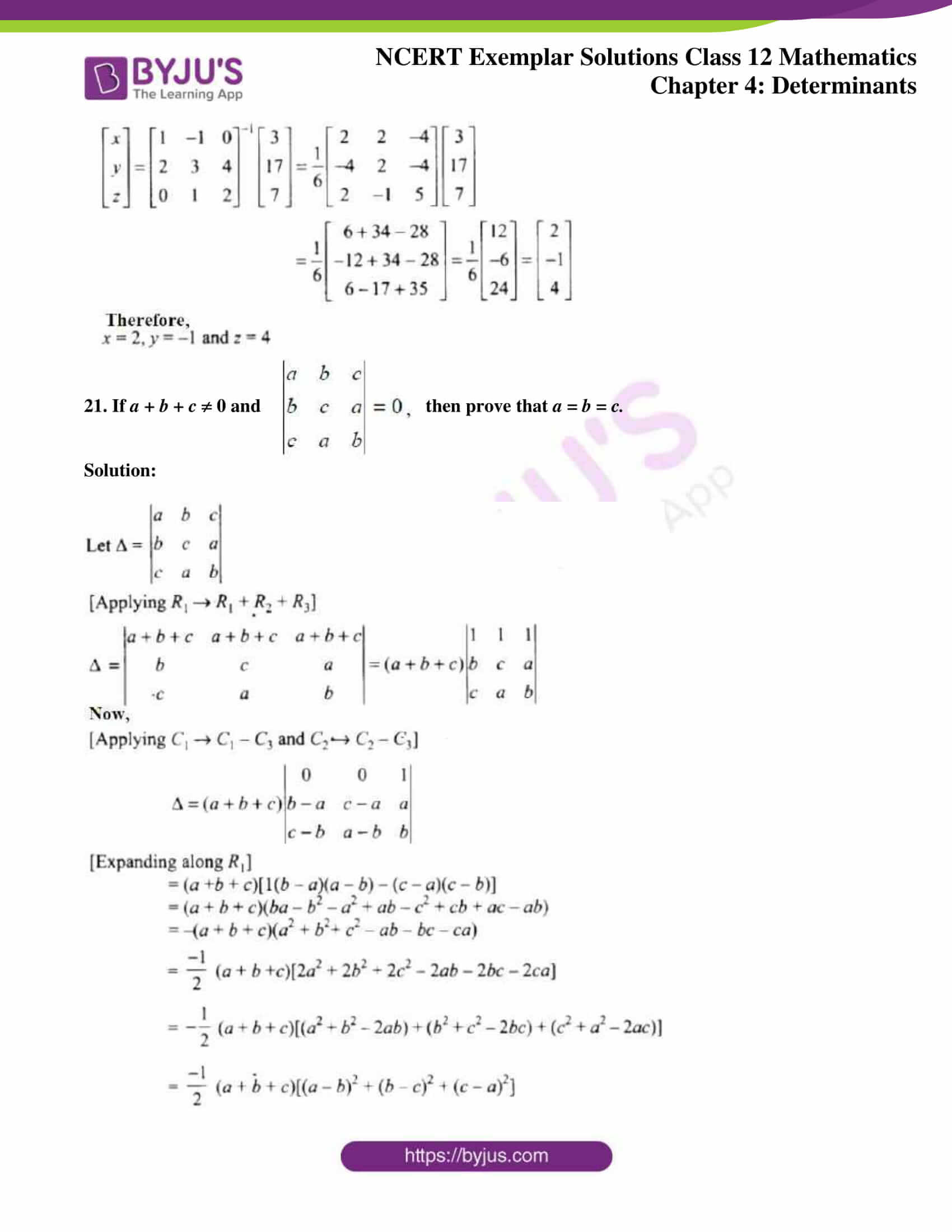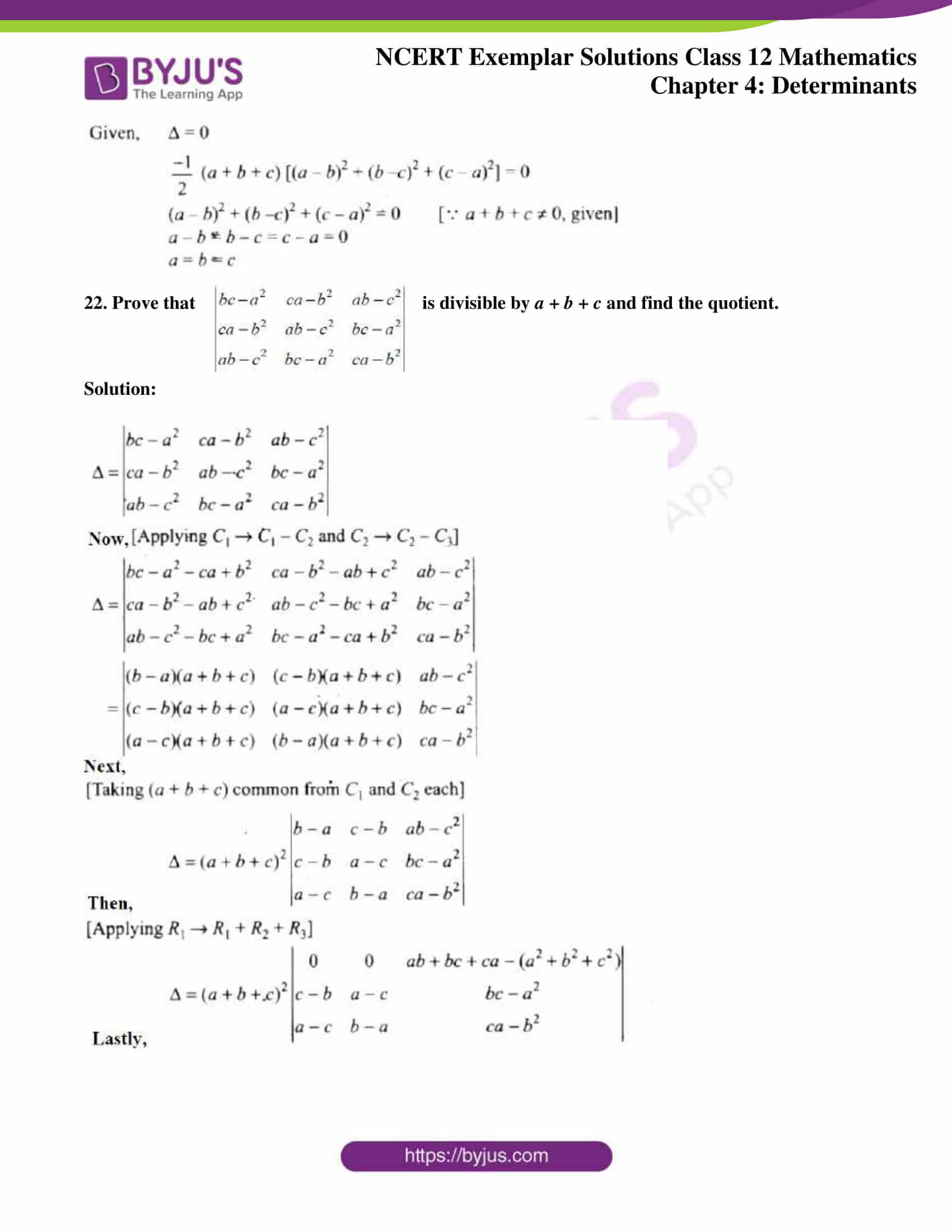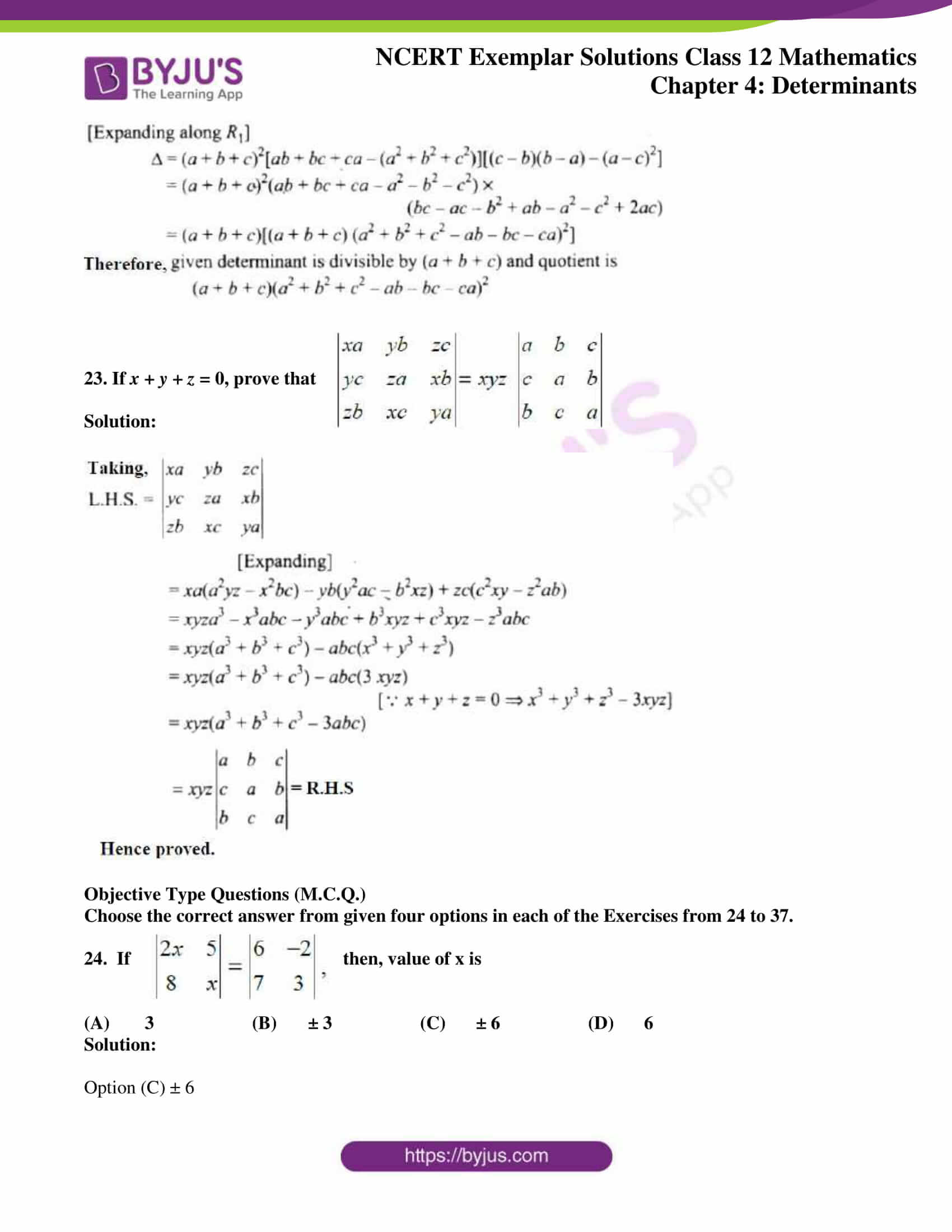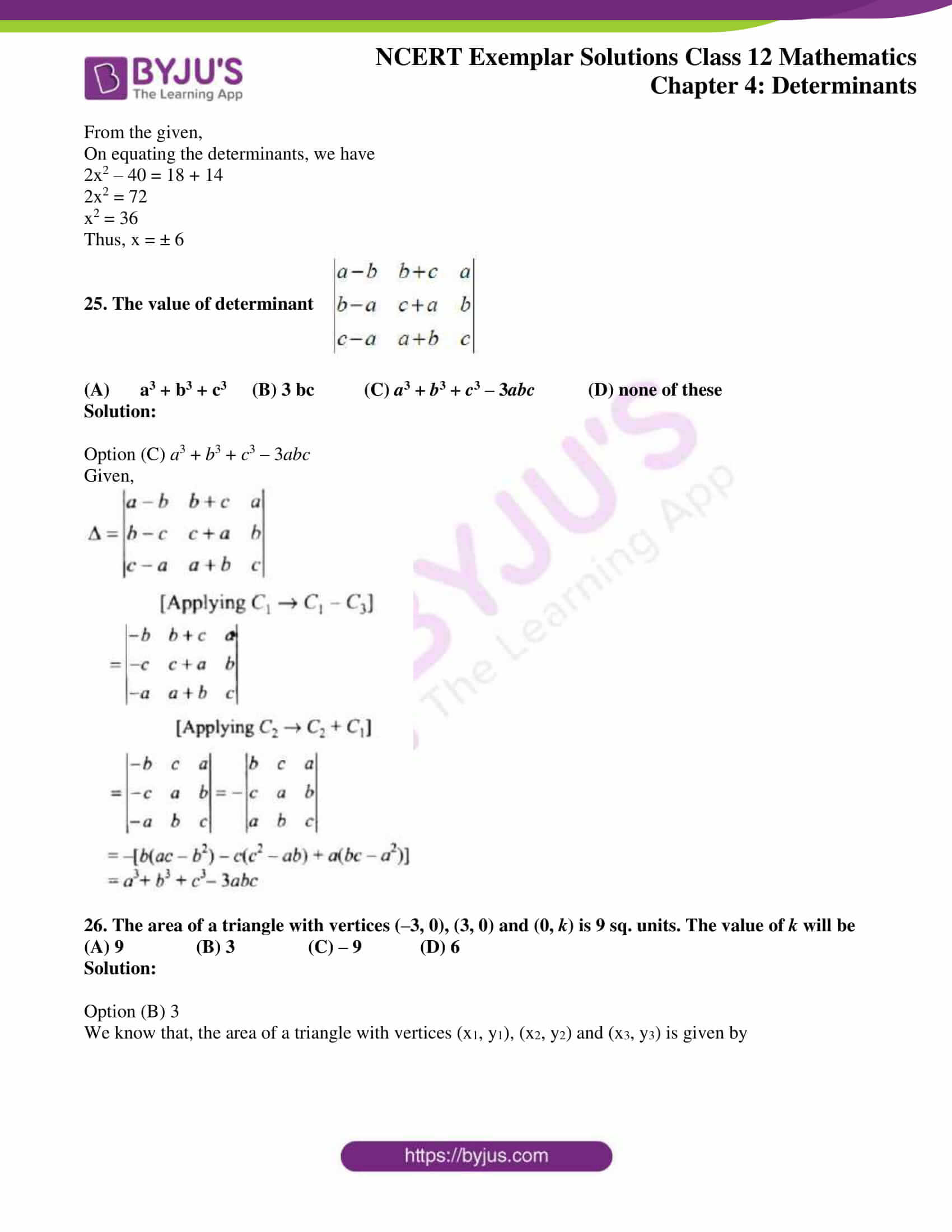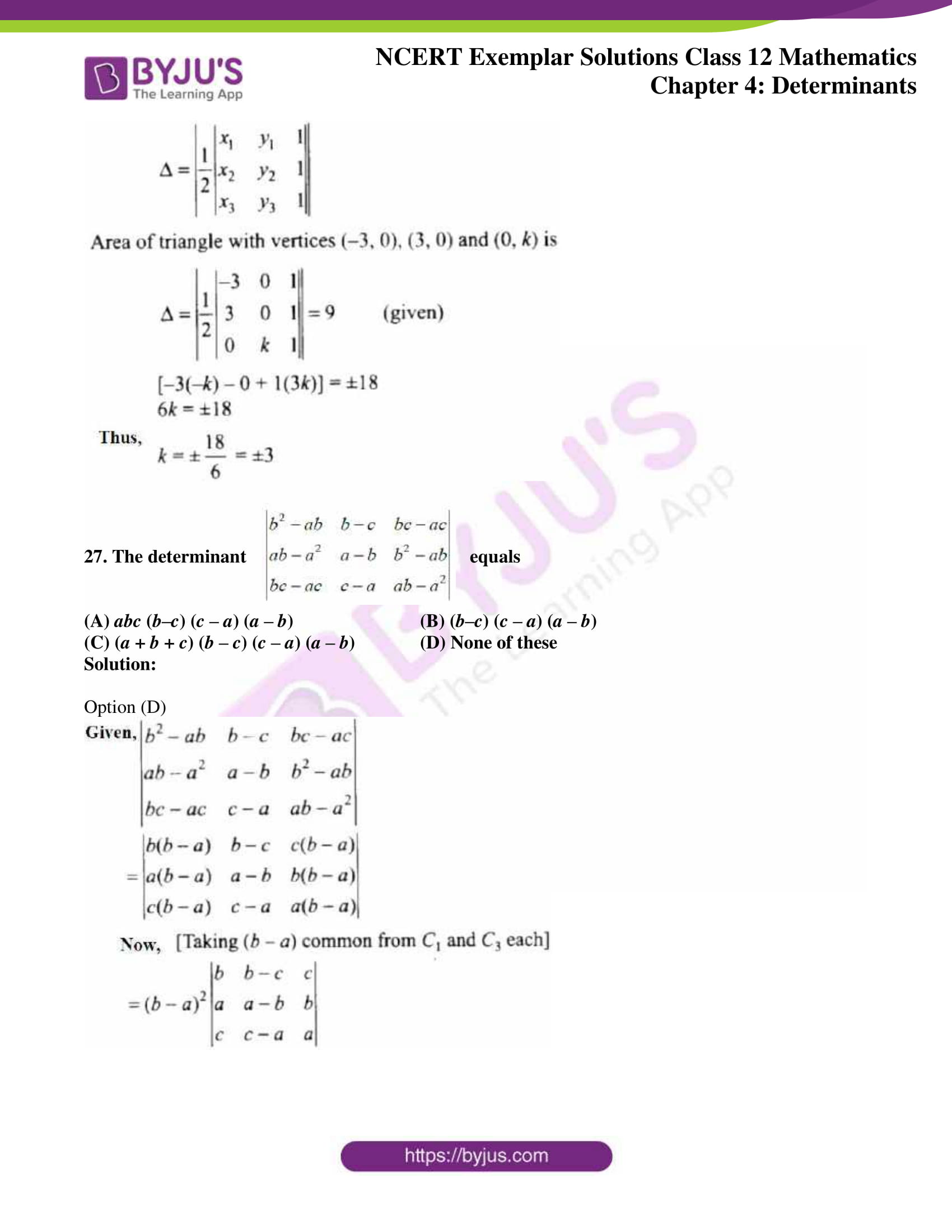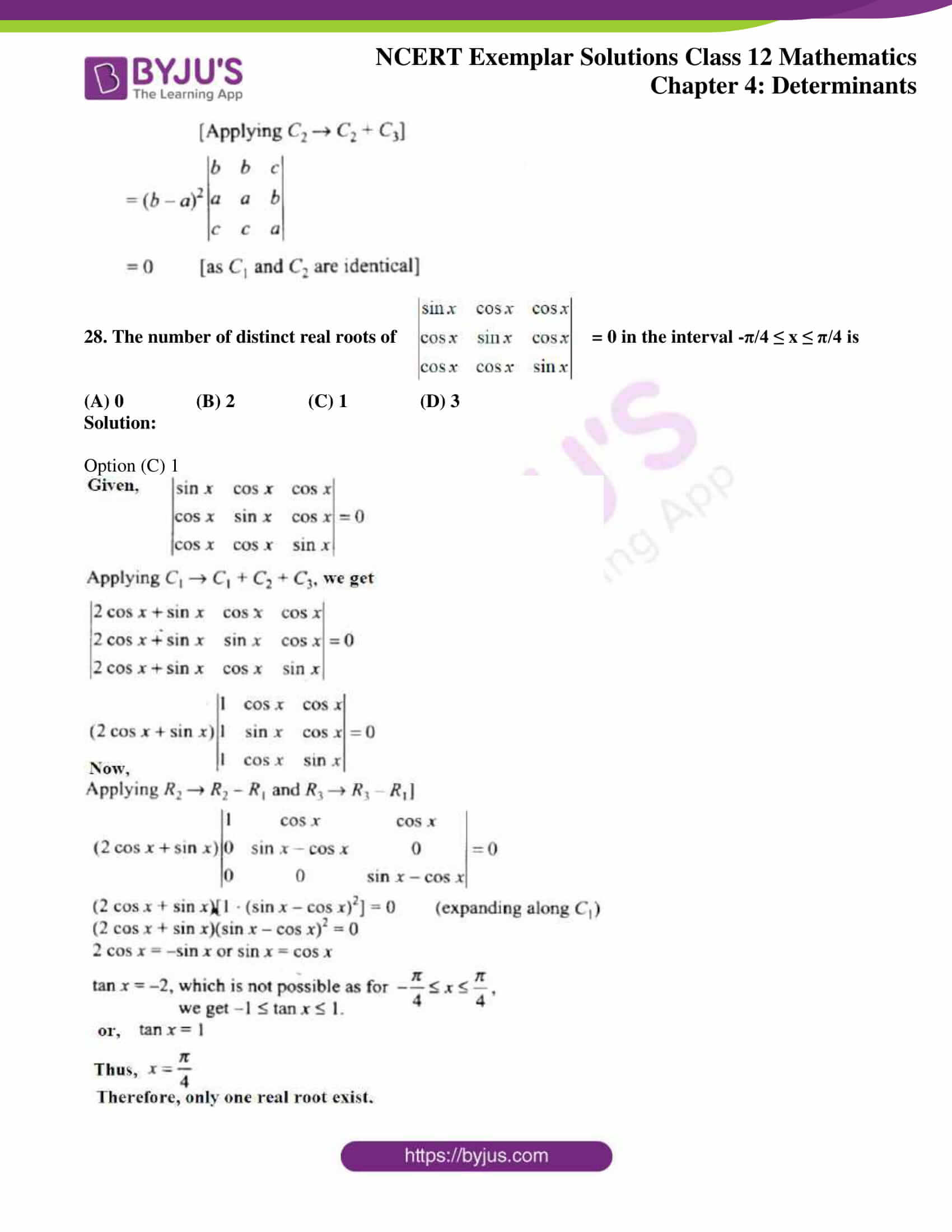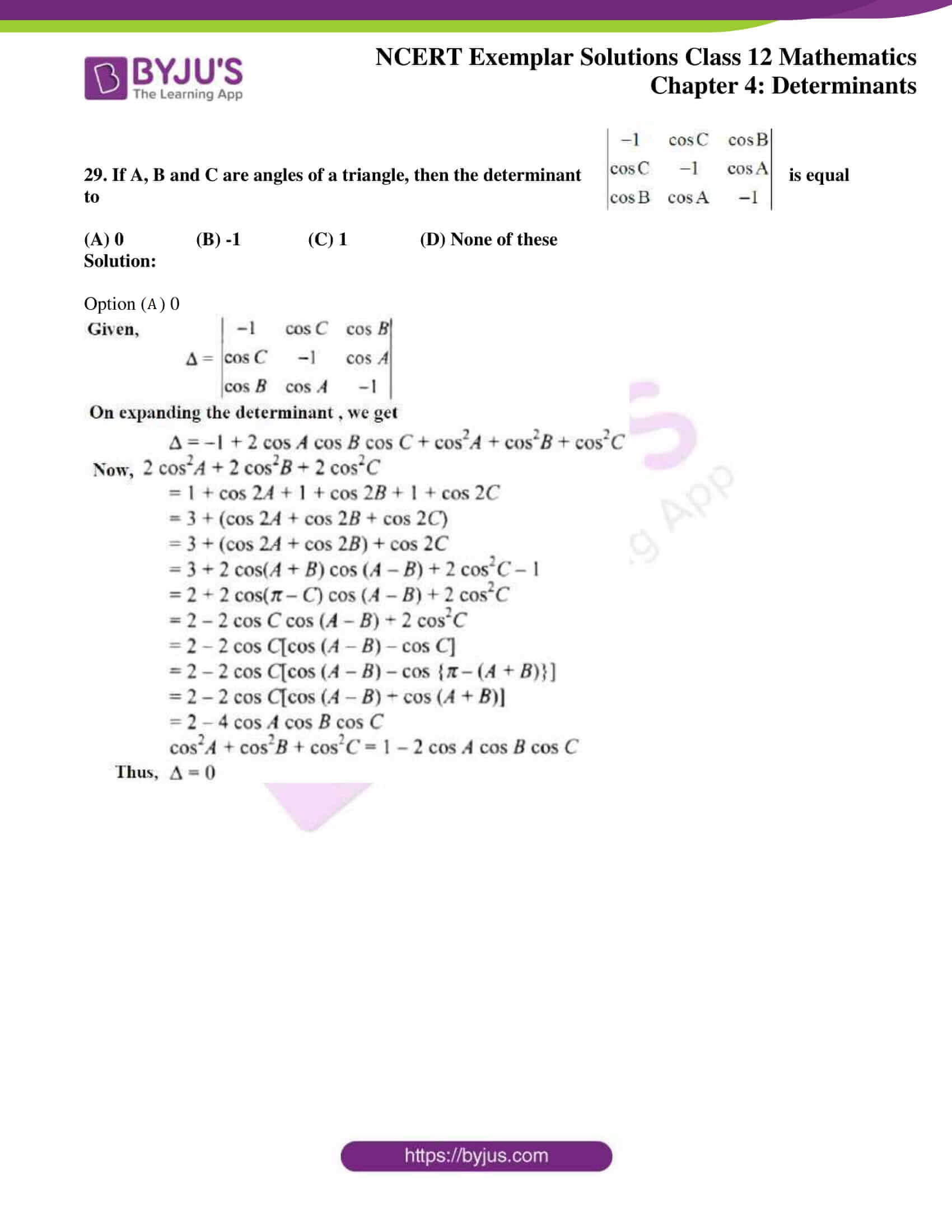### Access Answers to the NCERT Exemplar Class 12 Maths Chapter 4 Determinants

Exercise 4.3 Page No: 77

Using the properties of determinants in Exercises 1 to 6, evaluate:

1.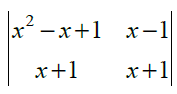Solution: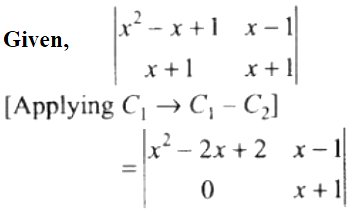= (x2 – 2x + 2) . (x + 1) – (x – 1) . 0

= x3 – 2x2 + 2x + x2 – 2x + 2

= x3 – x2 + 2

2.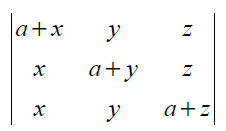Solution: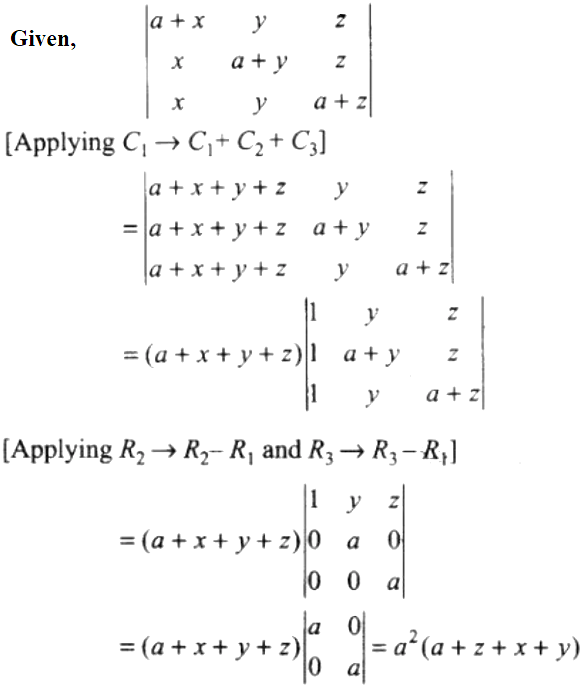3.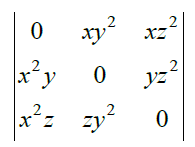Solution: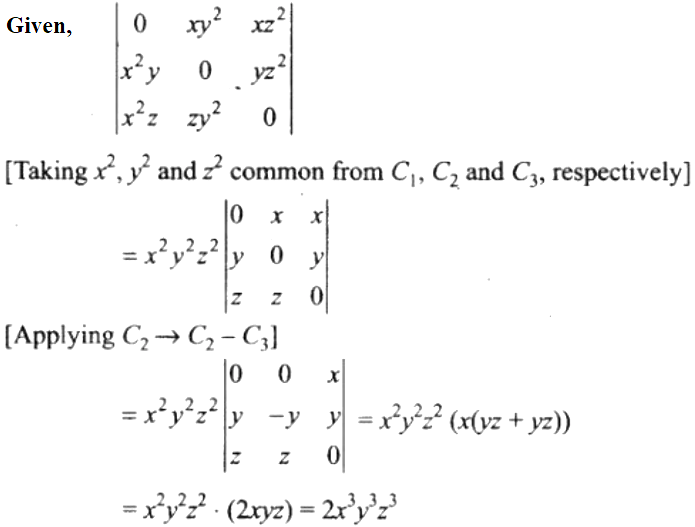4.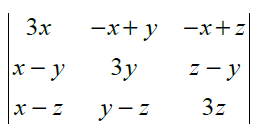Solution: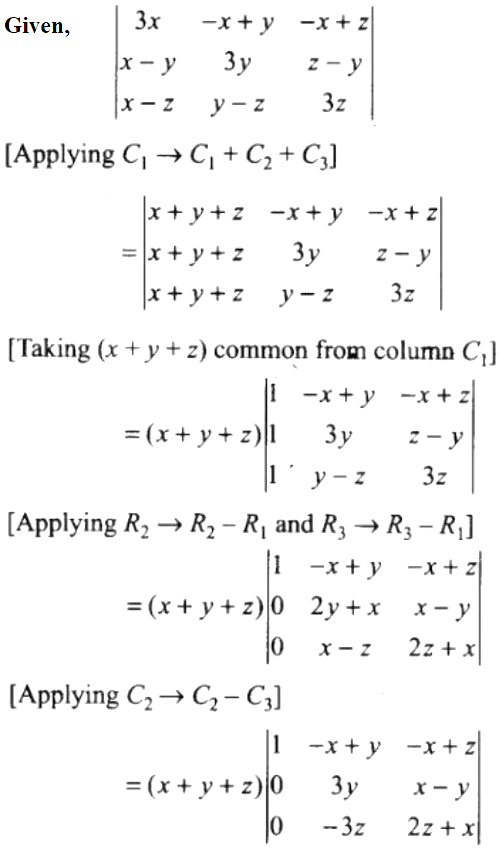[Expanding along first column]

= (x + y + z) . 1[3y(3z + x) + (3z)(x – y)]

= (x + y + z)(3yz + 3yx + 3xz)

= 3(x + y + z)(xy + yz + zx)

5.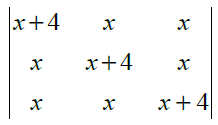Solution: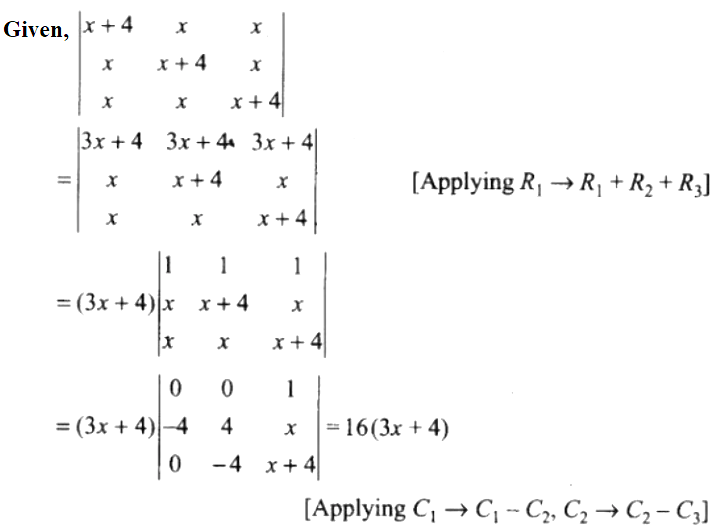6.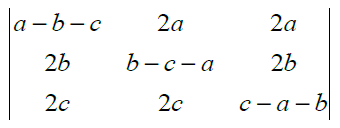Solution: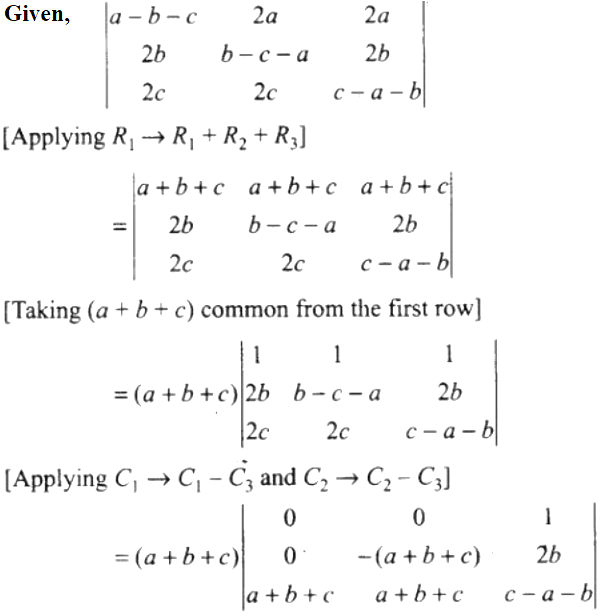Lastly, expanding along R1, we have

= (a + b + c) [1 x 0 + (a + b + c)2]

= (a + b + c)3

Using the properties of determinants in Exercises 7 to 9, prove that:

7.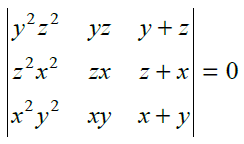Solution: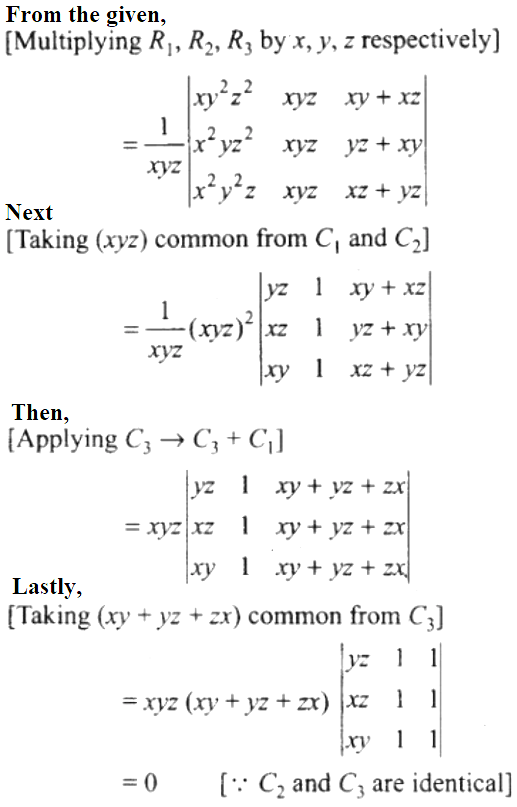8.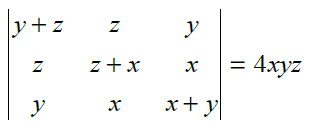Solution: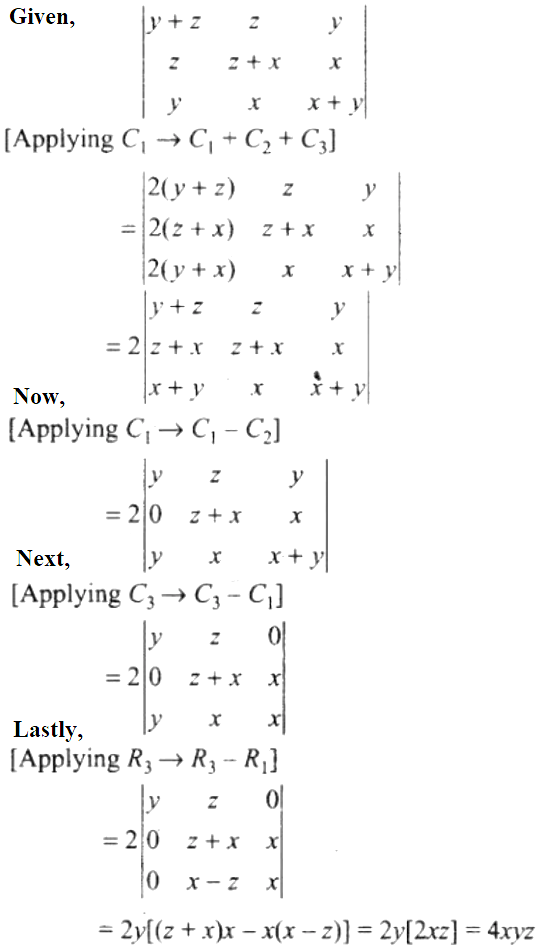9.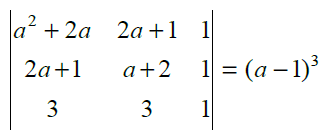Solution: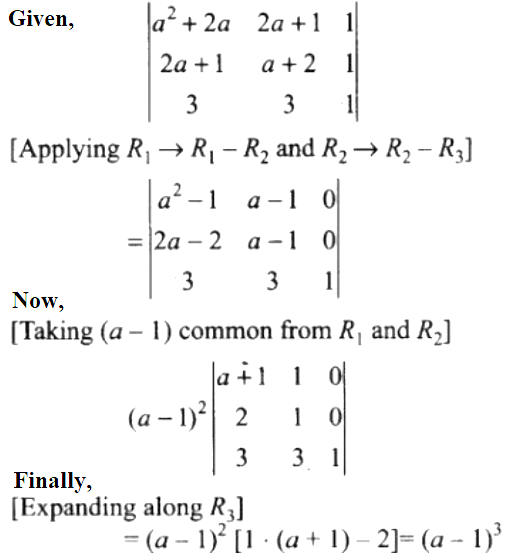10. If A + B + C = 0, then prove that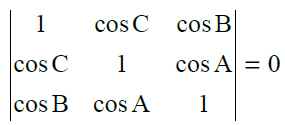Solution: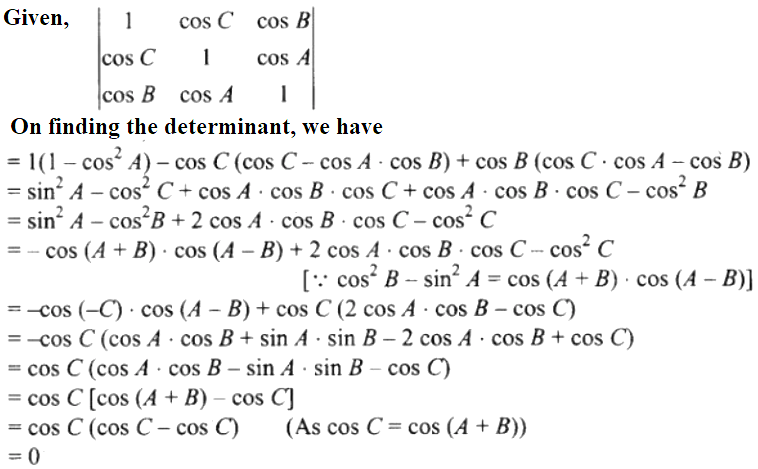11. If the co-ordinates of the vertices of an equilateral triangle with sides of length ‘a’ are (x1, y1),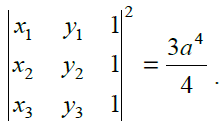(x2, y2), (x3, y3), then

Solution: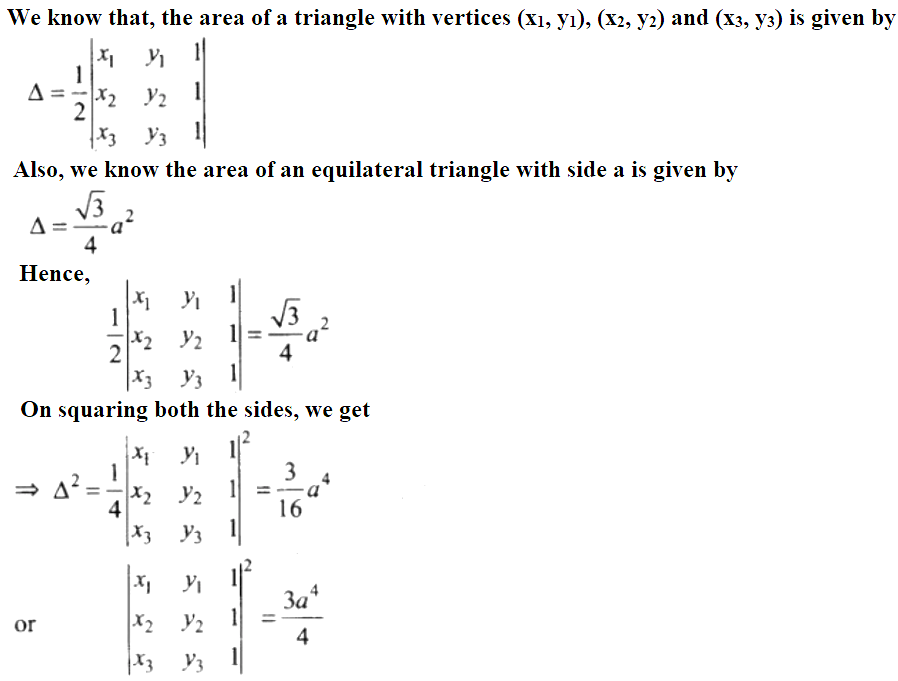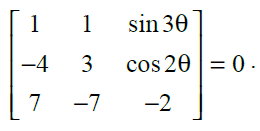12. Find the value of q satisfying

Solution: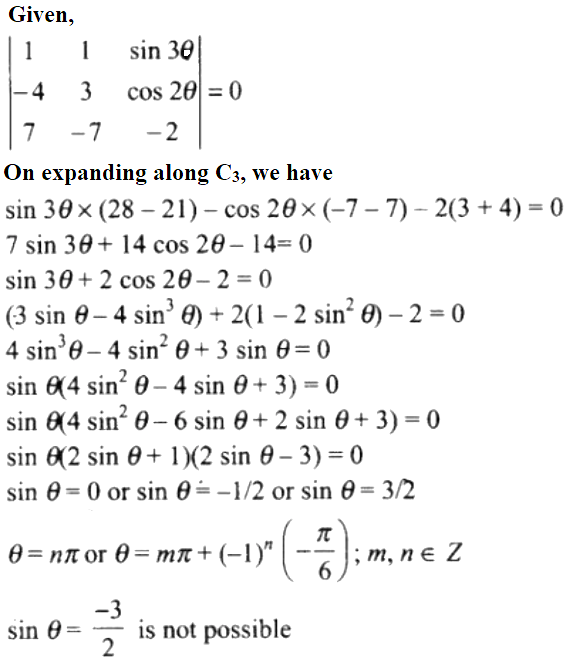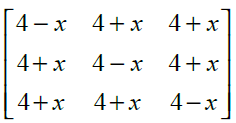13. If , then find values of x.

Solution: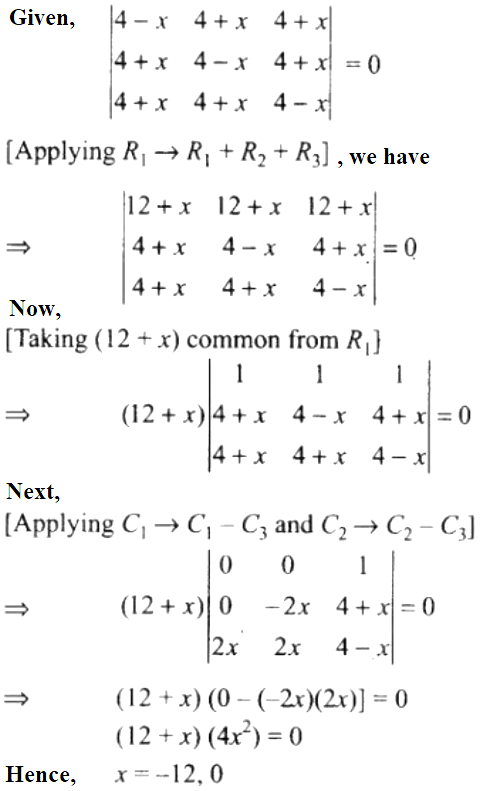14. If a1, a2, a3, …, ar are in G.P., then prove that the determinant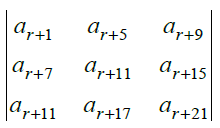is independent of r.

Solution: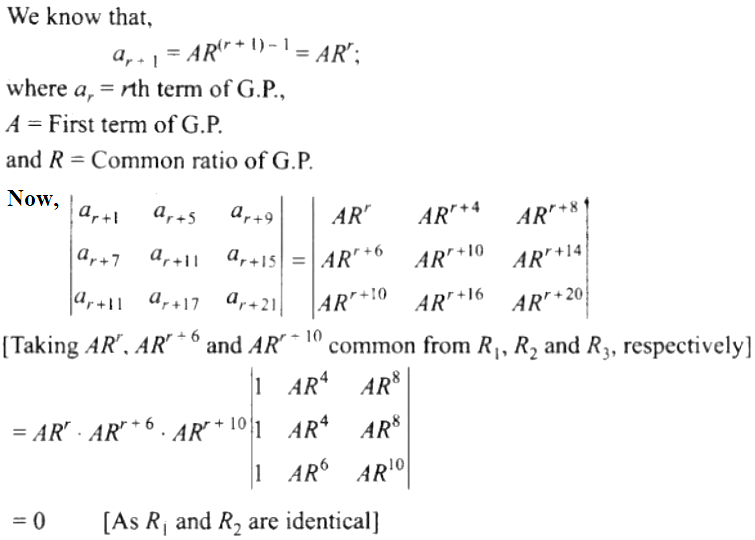Hence, the determinant is independent of r.

15. Show that the points (a + 5, a – 4), (a – 2, a + 3) and (a, a) do not lie on a straight line for any value of a.

Solution:

Given points are (a + 5, a – 4), (a – 2, a + 3) and (a, a).

Now, we have to prove that these points do not lie on a straight line.

So, if we prove that these points form a triangle then it can’t line on a straight line.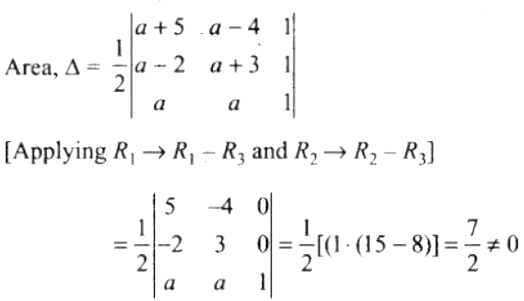Hence, the given points form a triangle and can’t lie on a straight line.

16. Show that the DABC is an isosceles triangle if the determinant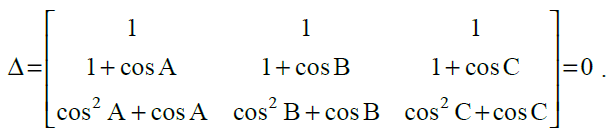Solution: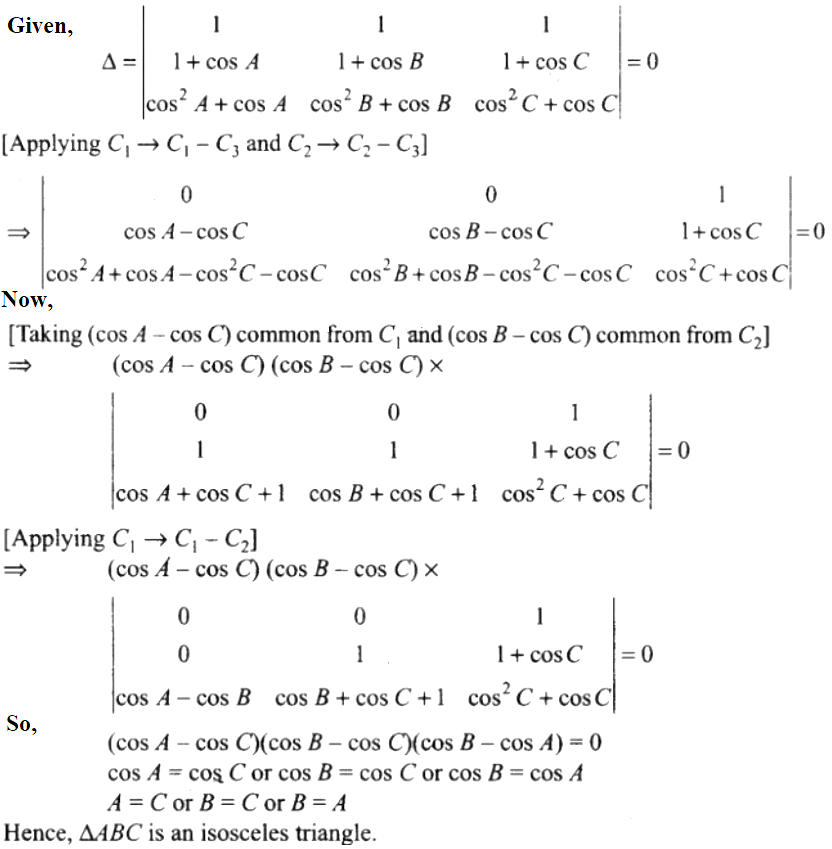17. Find A–1 if and show that A-1 = (A2 – 3I)/ 2.

Solution: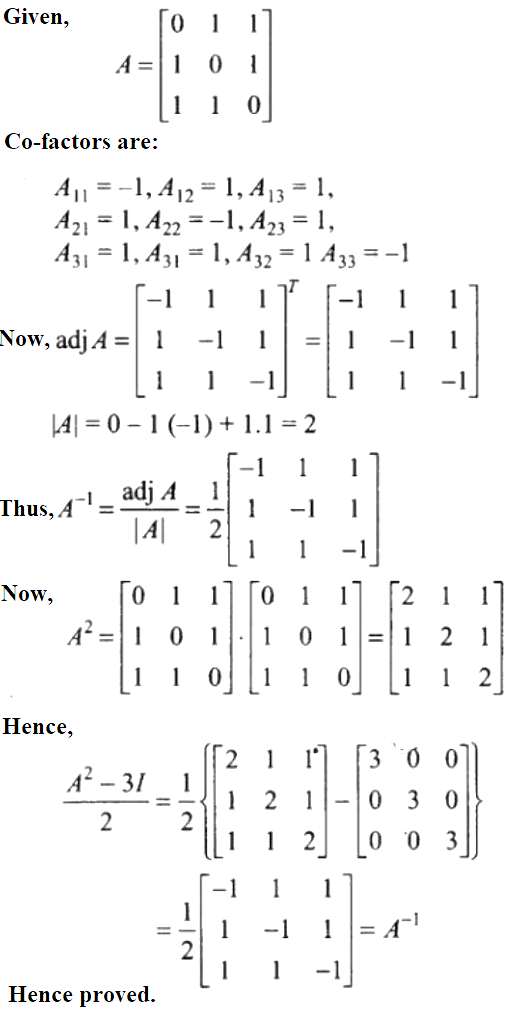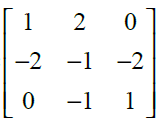18. If A = , find A-1.

Using A–1, solve the system of linear equations

x – 2y = 10 , 2x y z = 8 , –2y + z = 7.

Solution: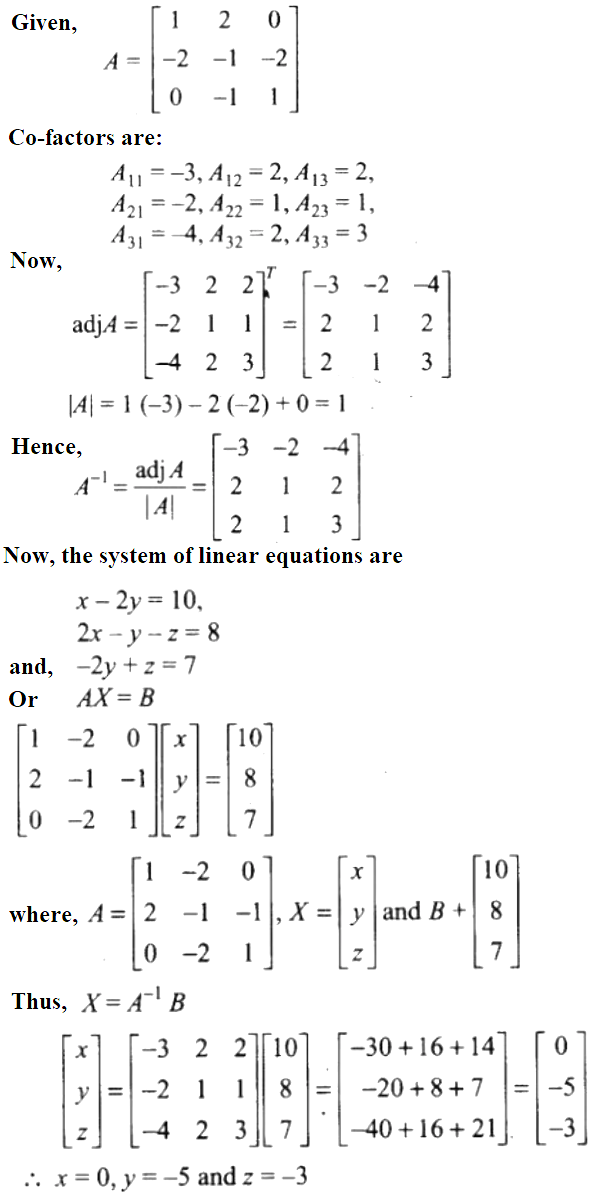19. Using matrix method, solve the system of equations

3x + 2y – 2z = 3, x + 2y + 3z = 6, 2x y + z = 2.

Solution:

Given system of equations are:

3x + 2y – 2z = 3

x + 2y + 3z = 6 and

2x y + z = 2

Or,

AX = B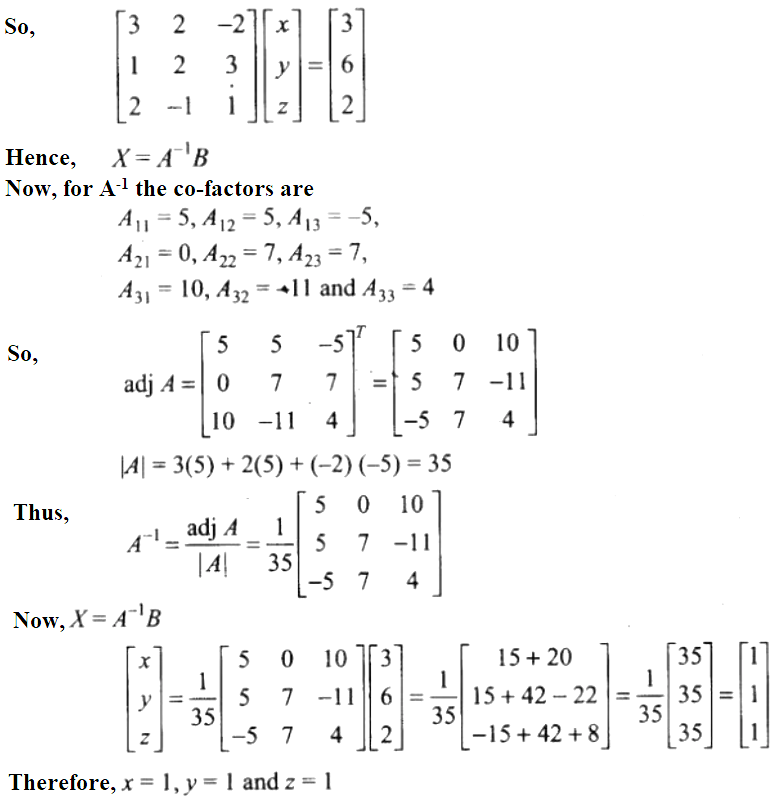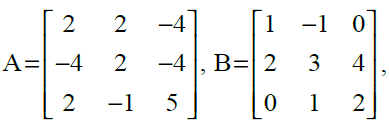20. Given find BA and use this to solve the system of

equations y + 2z = 7, x y = 3, 2x + 3y + 4z = 17.

Solution: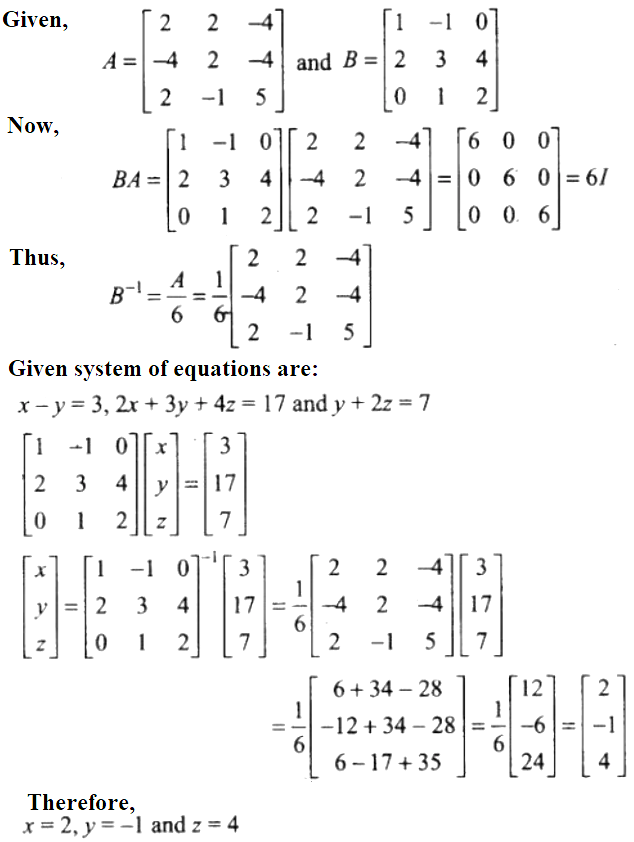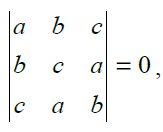21. If a + b + c ¹ 0 and then prove that a = b = c.

Solution: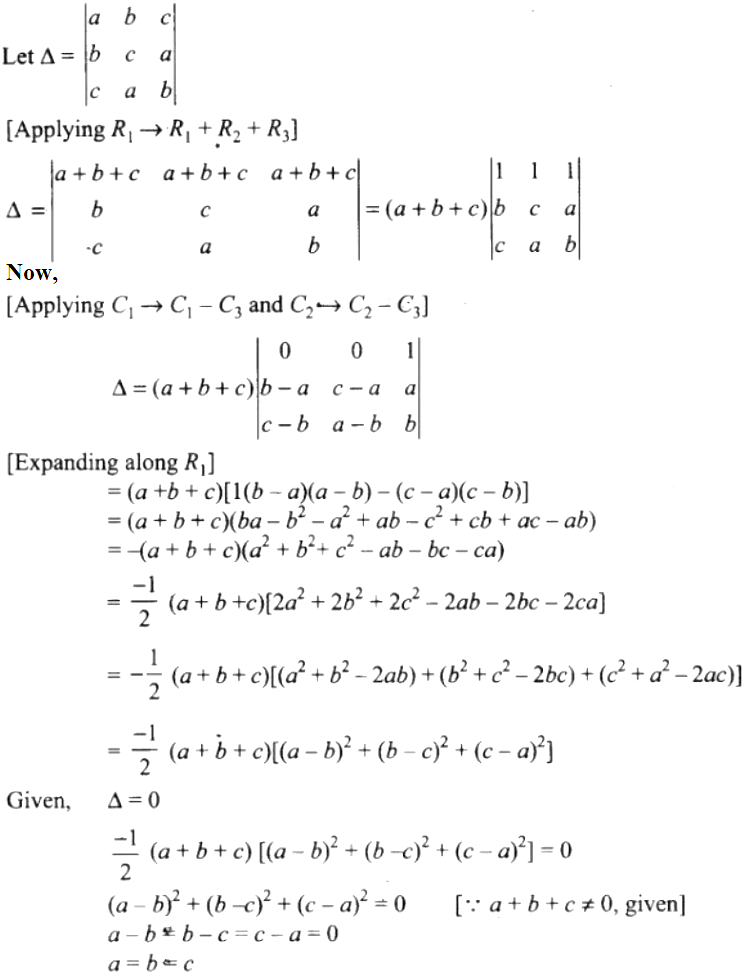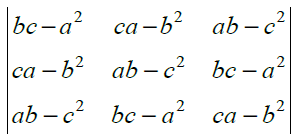22. Prove that is divisible by a + b + c and find the quotient.

Solution: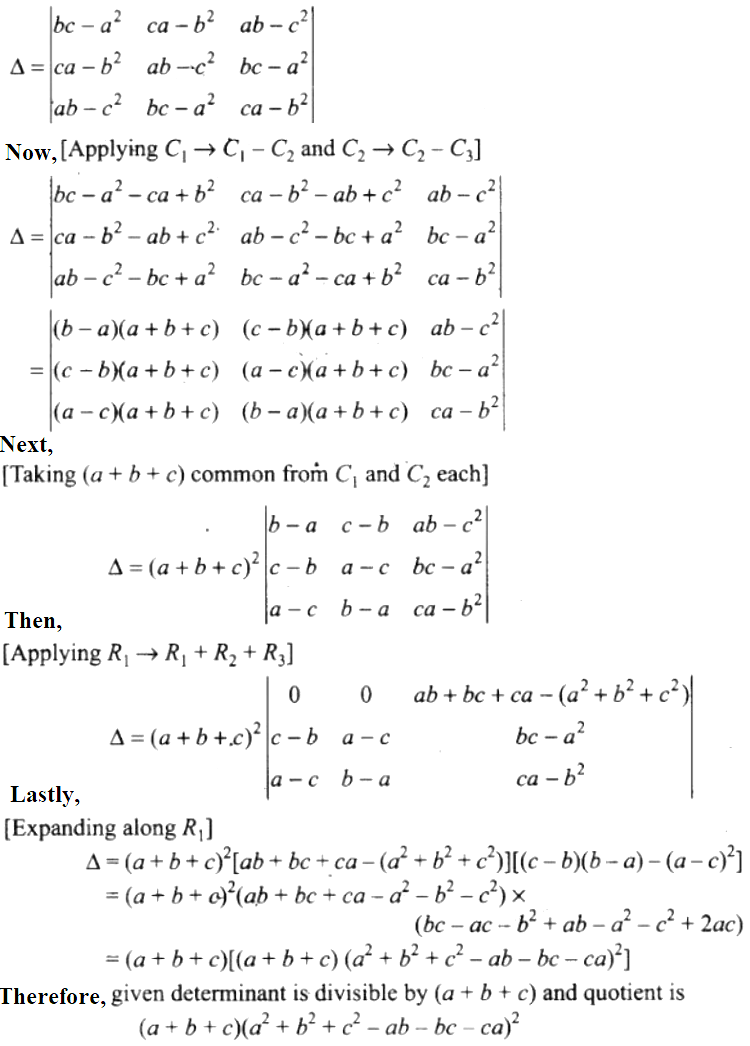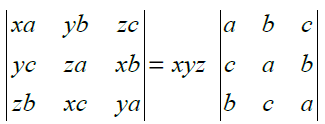23. If x + y + z = 0, prove that

Solution: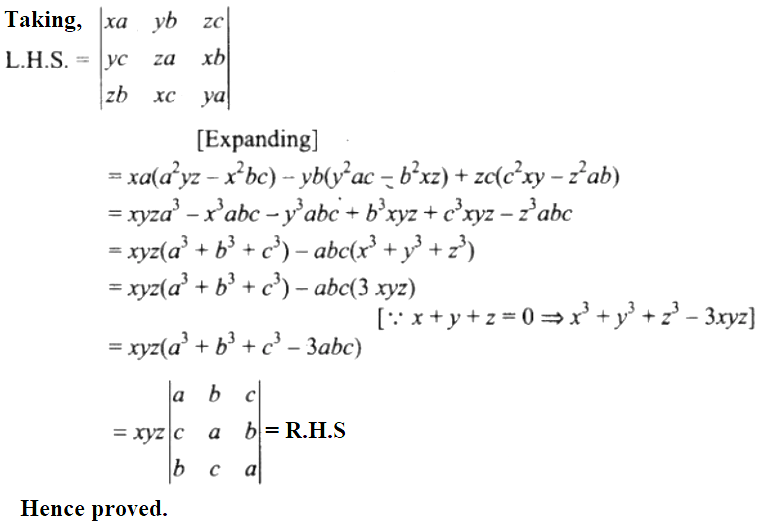Objective Type Questions (M.C.Q.)

Choose the correct answer from given four options in each of the Exercises from 24 to 37.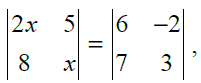24. If then, value of x is

(A) 3 (B) ± 3 (C) ± 6 (D) 6

Solution:

Option (C) ± 6

From the given,

On equating the determinants, we have

2x2 – 40 = 18 + 14

2x2 = 72

x2 = 36

Thus, x = ± 6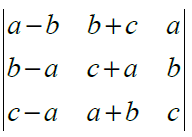25. The value of determinant

(A) a3 + b3 + c3 (B) 3 bc (C) a3 + b3 + c3 – 3abc (D) none of these

Solution:

Option (C) a3 + b3 + c3 – 3abc

Given,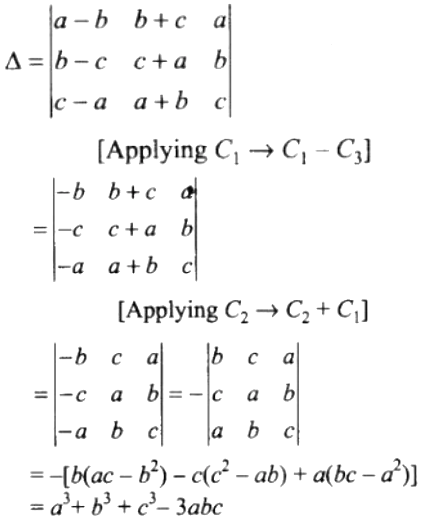26. The area of a triangle with vertices (–3, 0), (3, 0) and (0, k) is 9 sq. units. The value of k will be

(A) 9 (B) 3 (C) – 9 (D) 6

Solution:

Option (B) 3

We know that, the area of a triangle with vertices (x1, y1), (x2, y2) and (x3, y3) is given by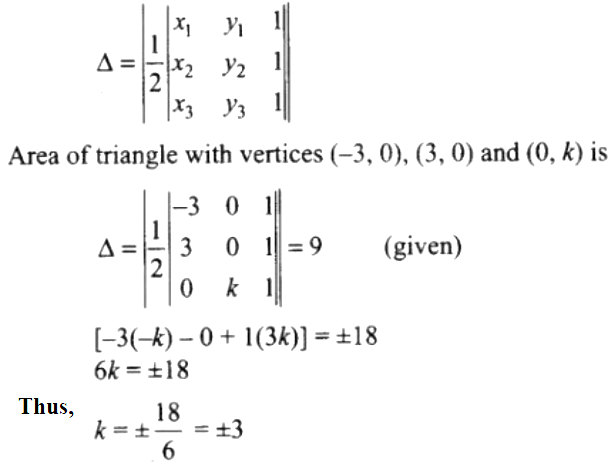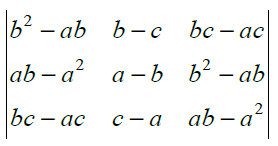27. The determinant equals

(A) abc (bc) (c a) (a b) (B) (bc) (c a) (a b)

(C) (a + b + c) (b c) (c a) (a b) (D) None of these

Solution:

Option (D)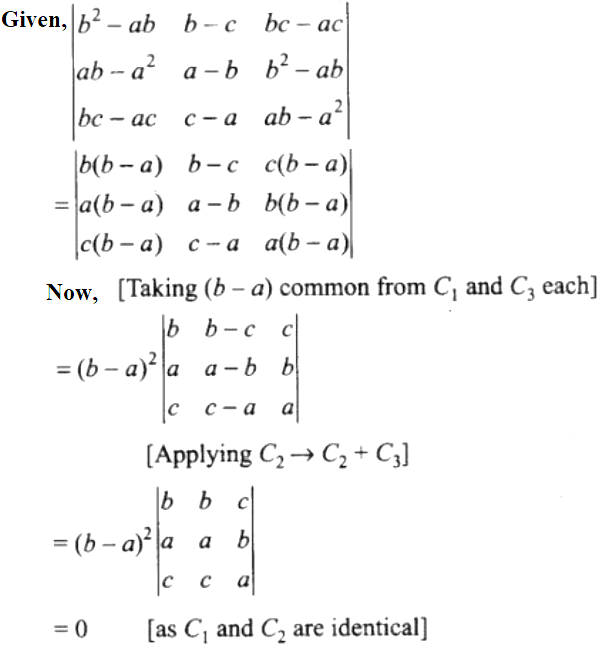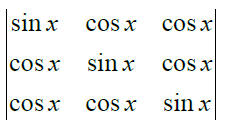28. The number of distinct real roots of = 0 in the interval -π/4 ≤ x ≤ π/4 is

(A) 0 (B) 2 (C) 1 (D) 3

Solution:

Option (C) 1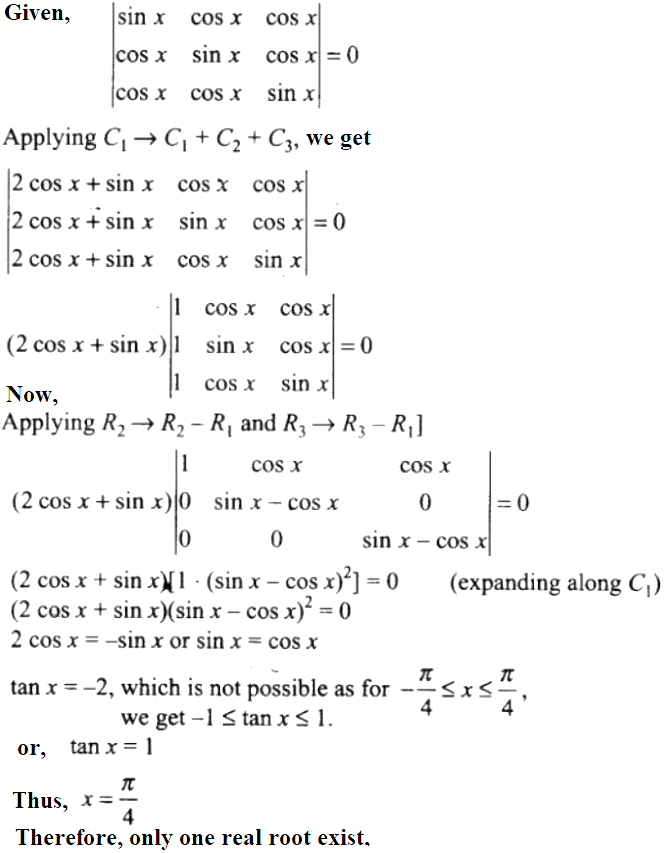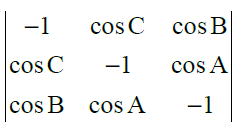29. If A, B and C are angles of a triangle, then the determinant is equal to

(A) 0 (B) -1 (C) 1 (D) None of these

Solution:

Option (A) 0